# Selina Solutions Concise Maths Class 10 Chapter 15 Similarity (With Applications to Maps and Models)

When two figures have the same shape but differ in size then they are said to be similar figures. This Chapter deals with the similarity of triangles. Basics of understanding corresponding sides and corresponding angles of similar triangles, various conditions for similarity of two triangles, Basic Proportionality theorem, relation between the areas of two triangles, similarity as a size transformation and finally the applications to maps and models are the key concepts covered under this Chapter. Students who find it difficult to solve problems in this Chapter or others can refer to Selina Solutions for Class 10 Mathematics, which are prepared by experienced faculty members at BYJU’S. This is created with a purpose to build confidence among students, who are preparing for their ICSE examinations. The Selina Solutions Concise Maths Class 10 Chapter 15 Similarity (With Applications to Maps and Models) PDF is available in the link below.

## Selina Solutions Concise Maths Class 10 Chapter 15 Similarity (With Applications to Maps and Models) Download PDF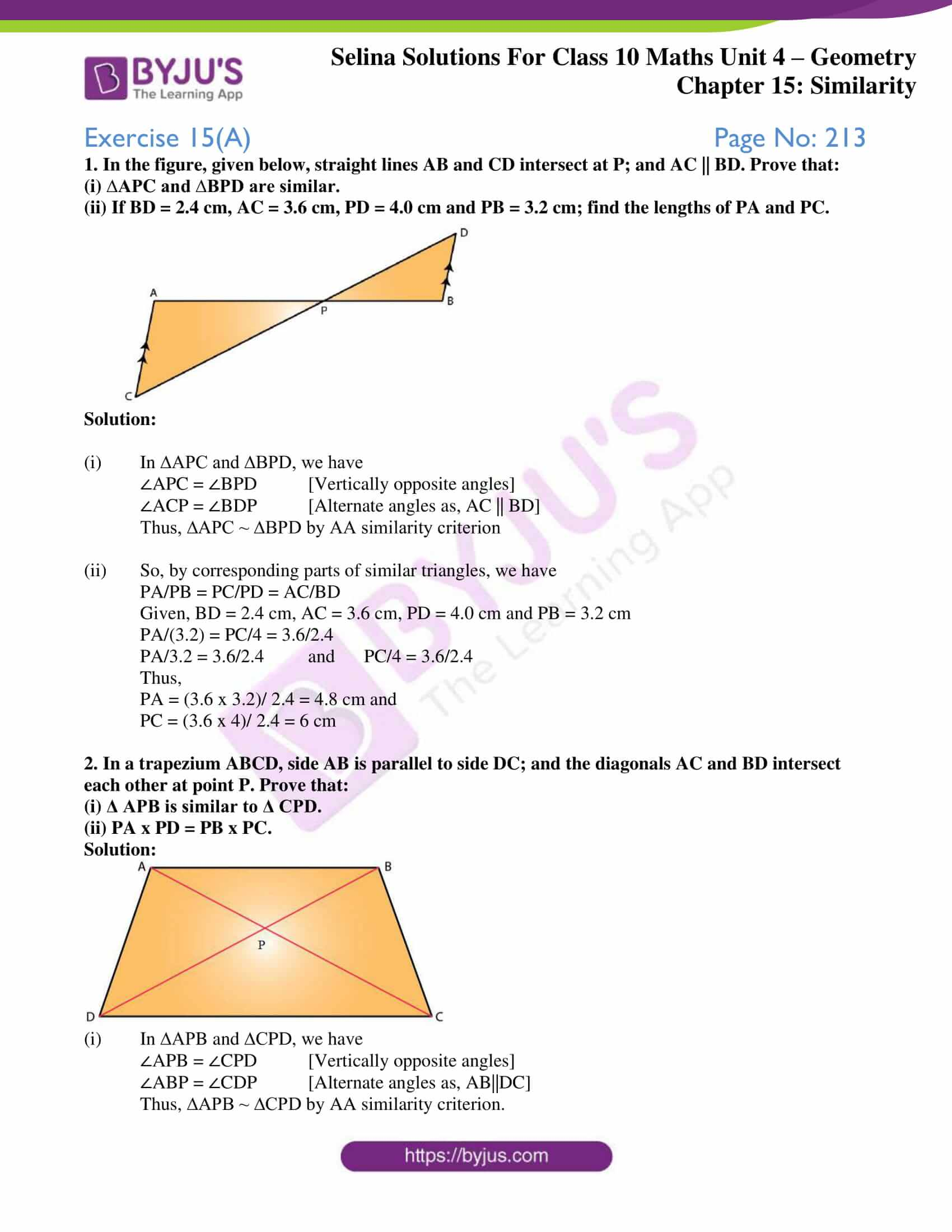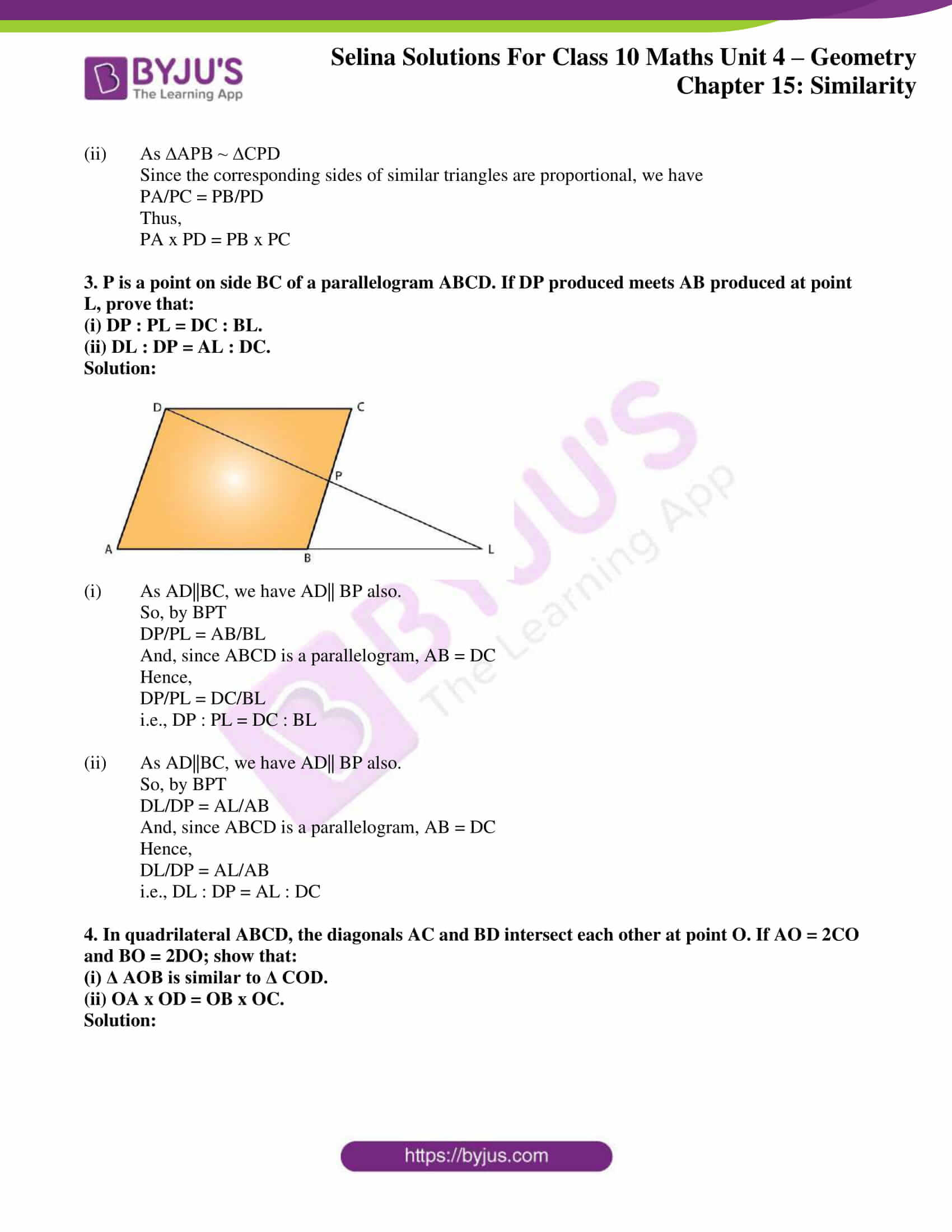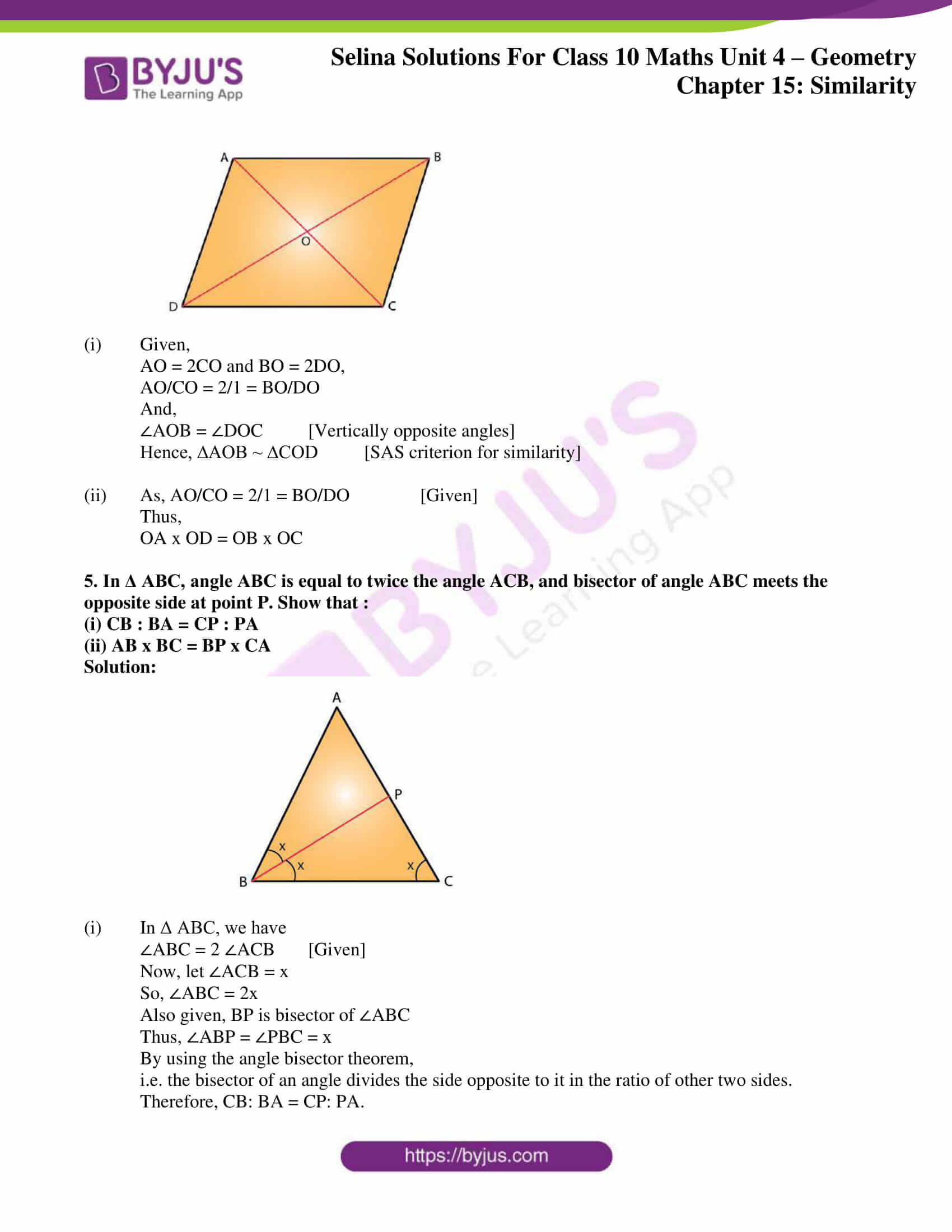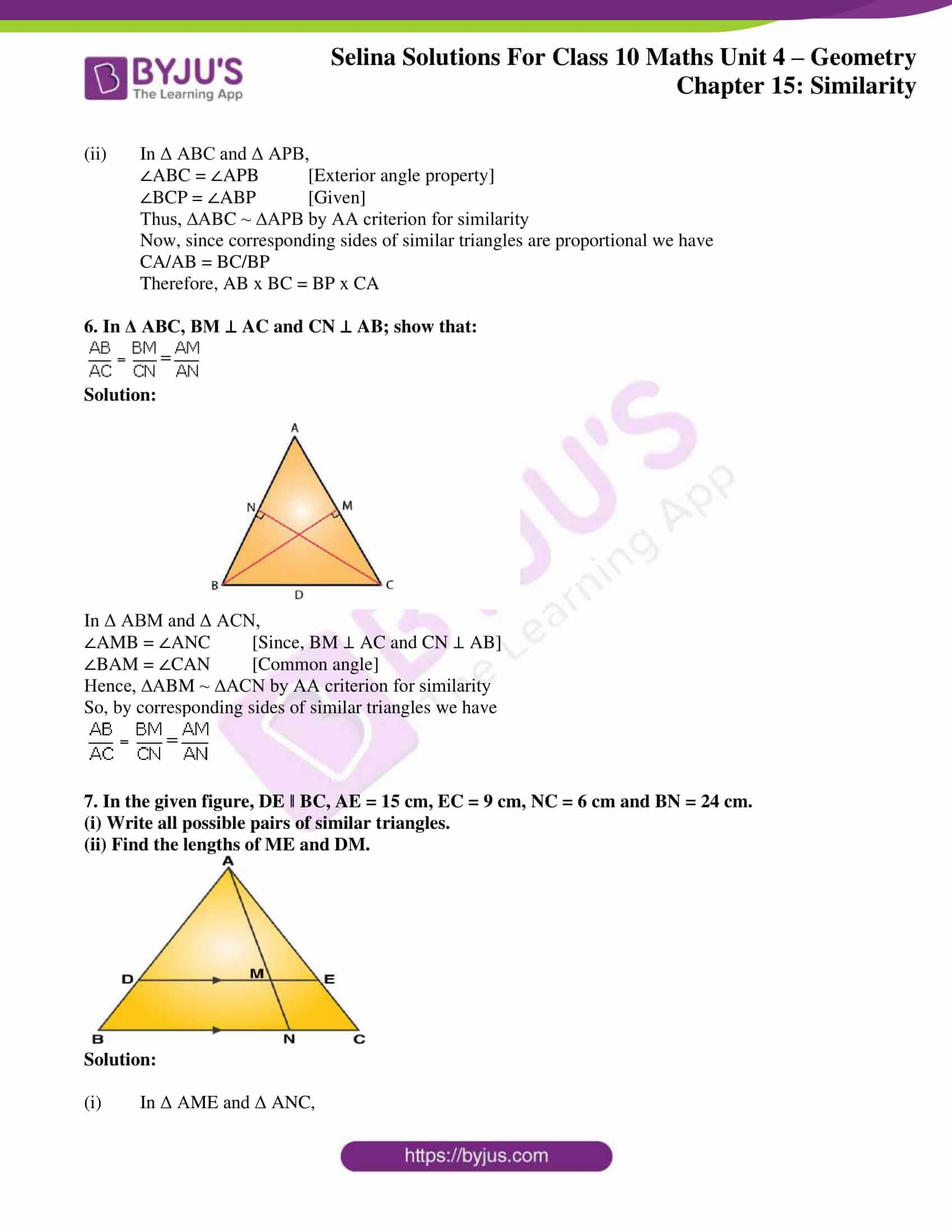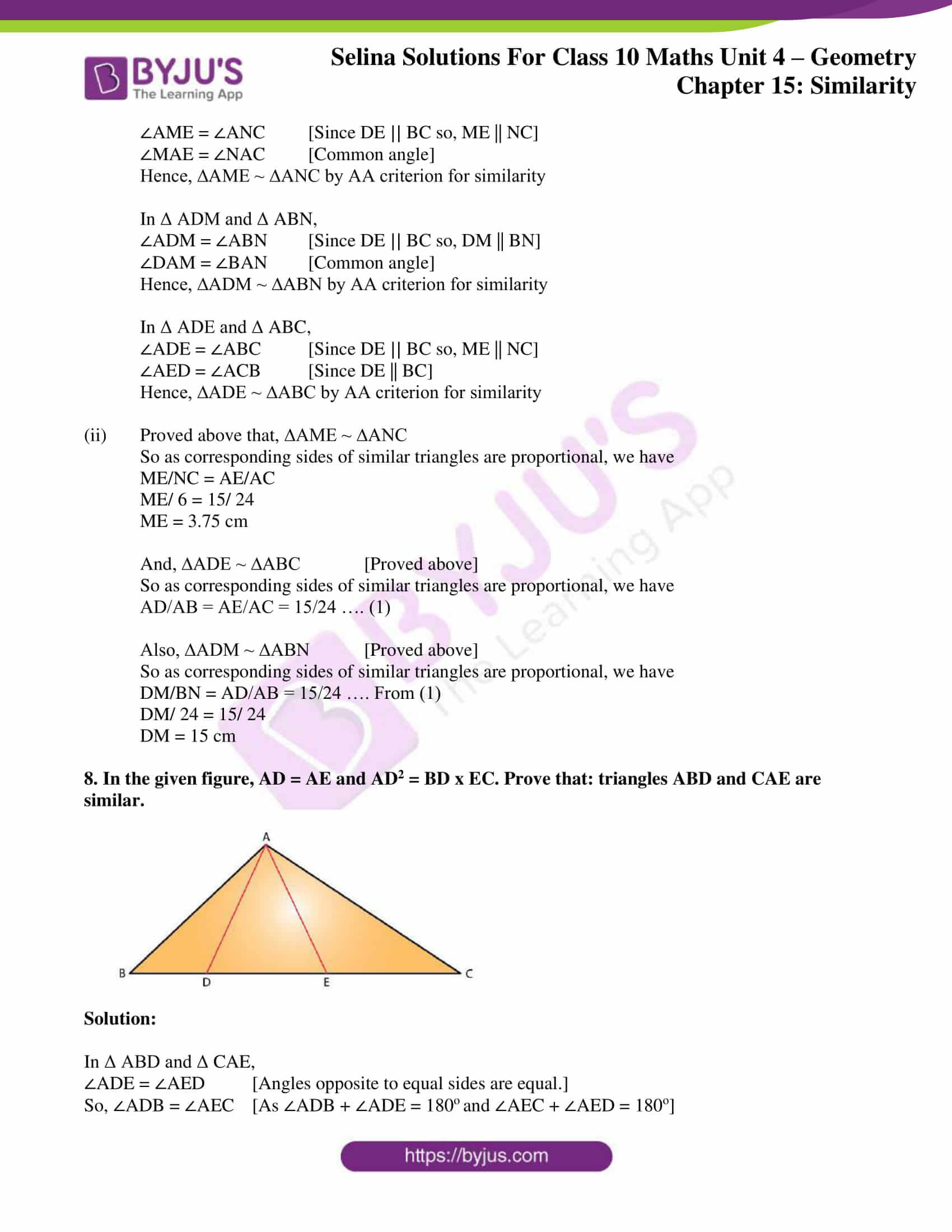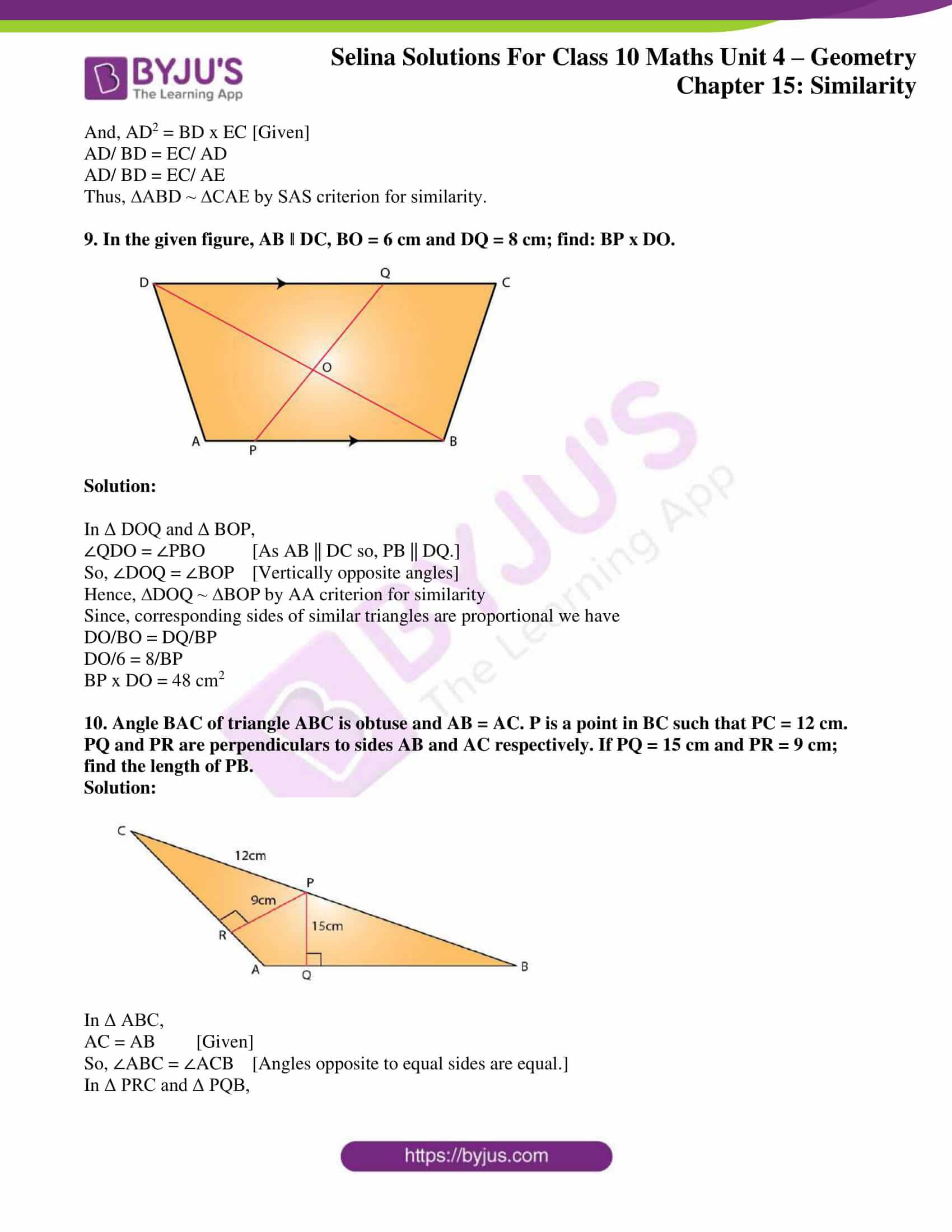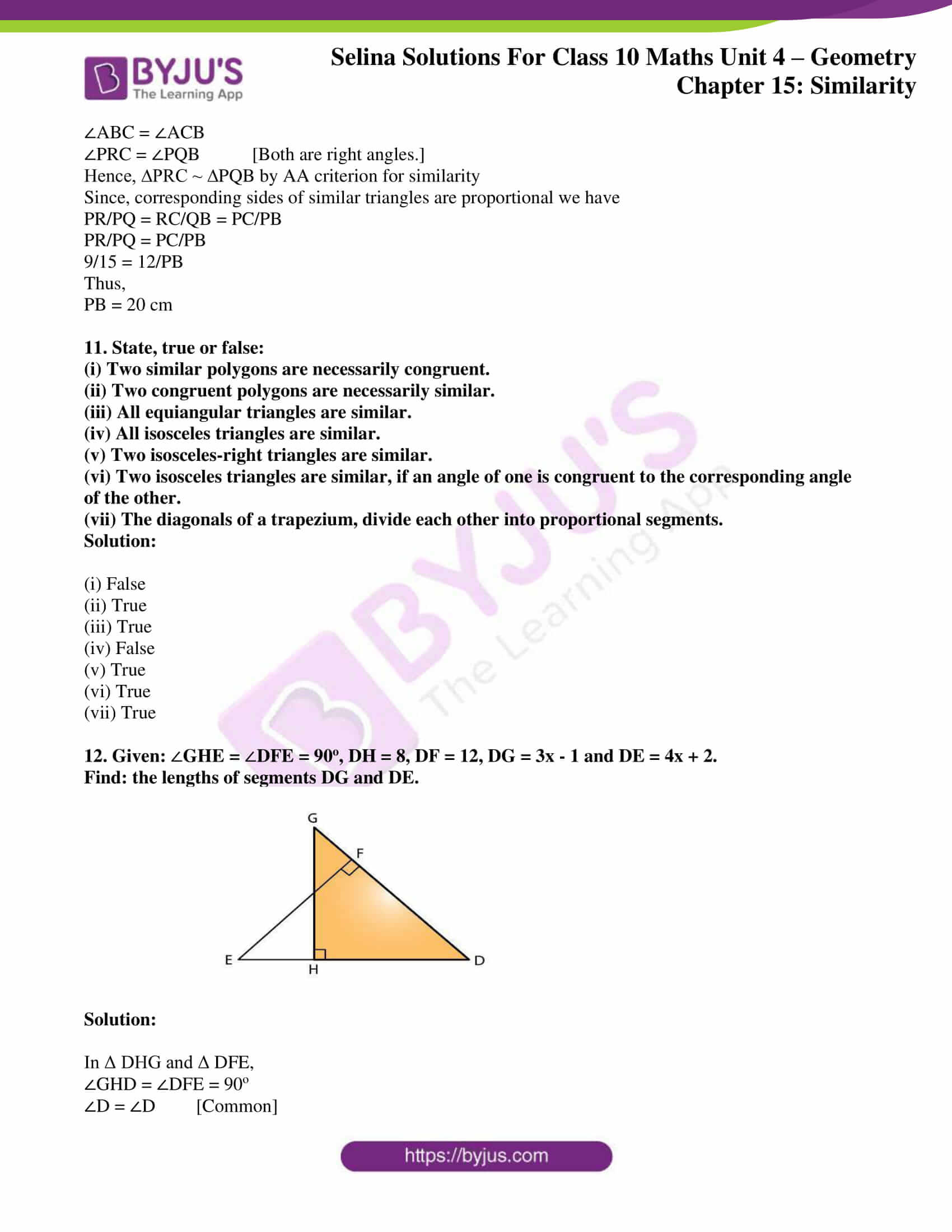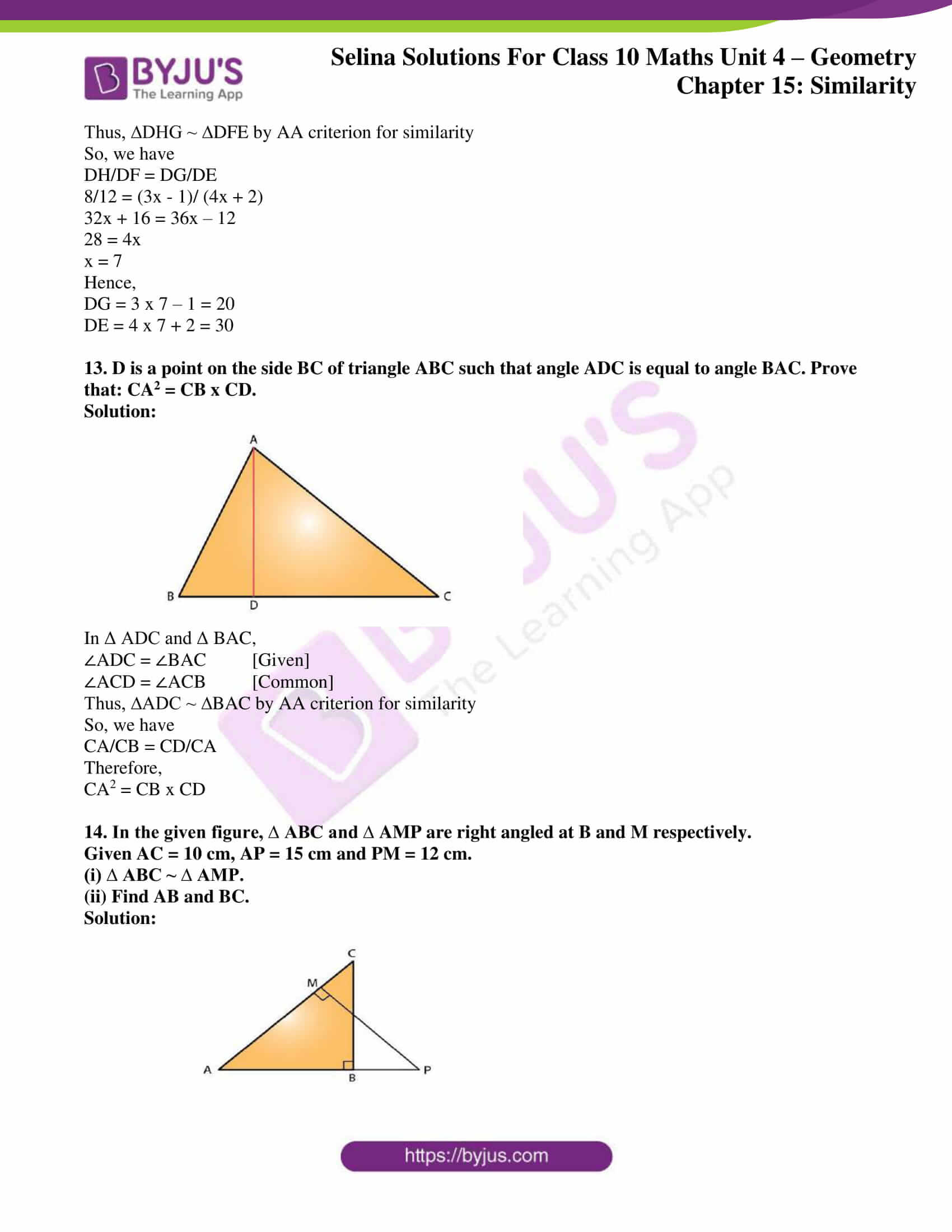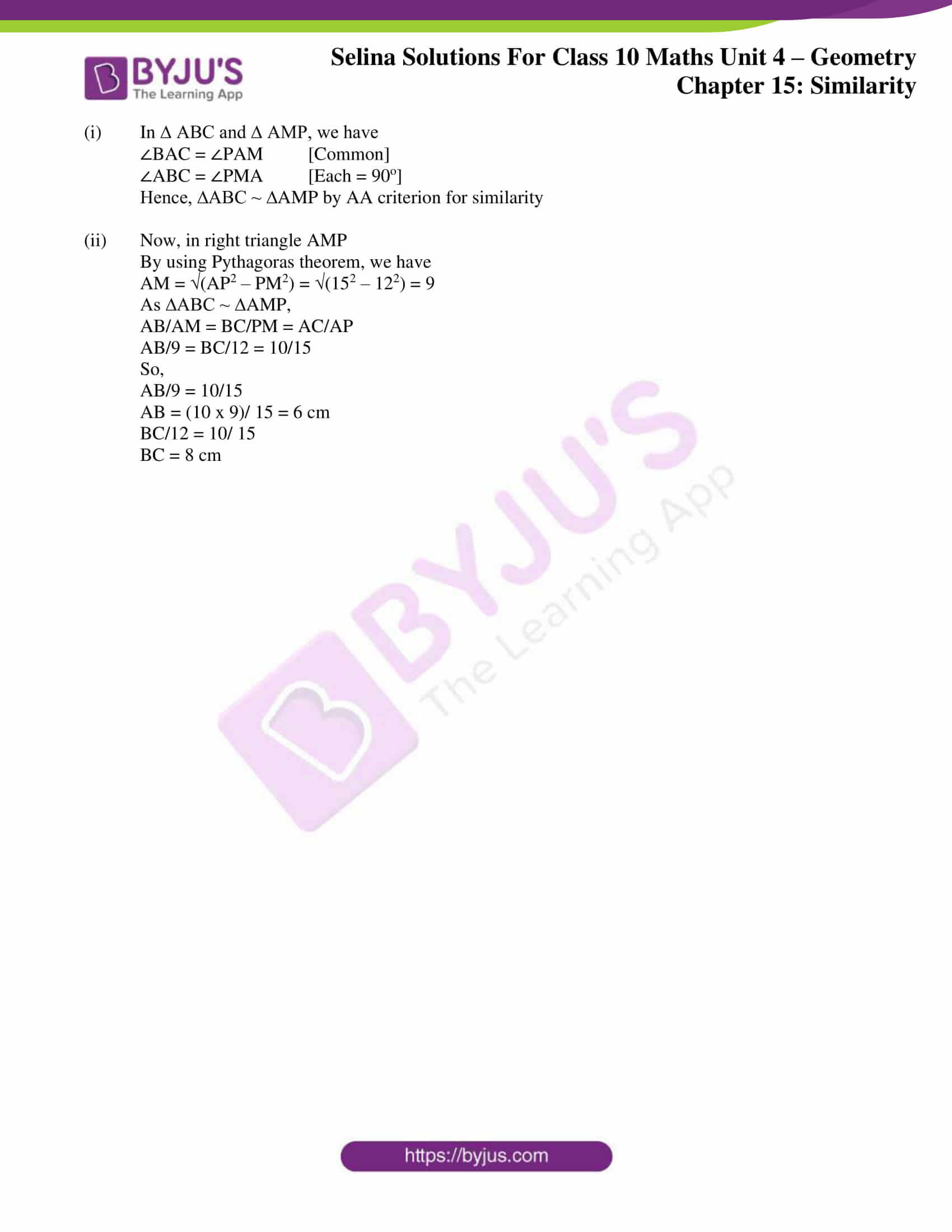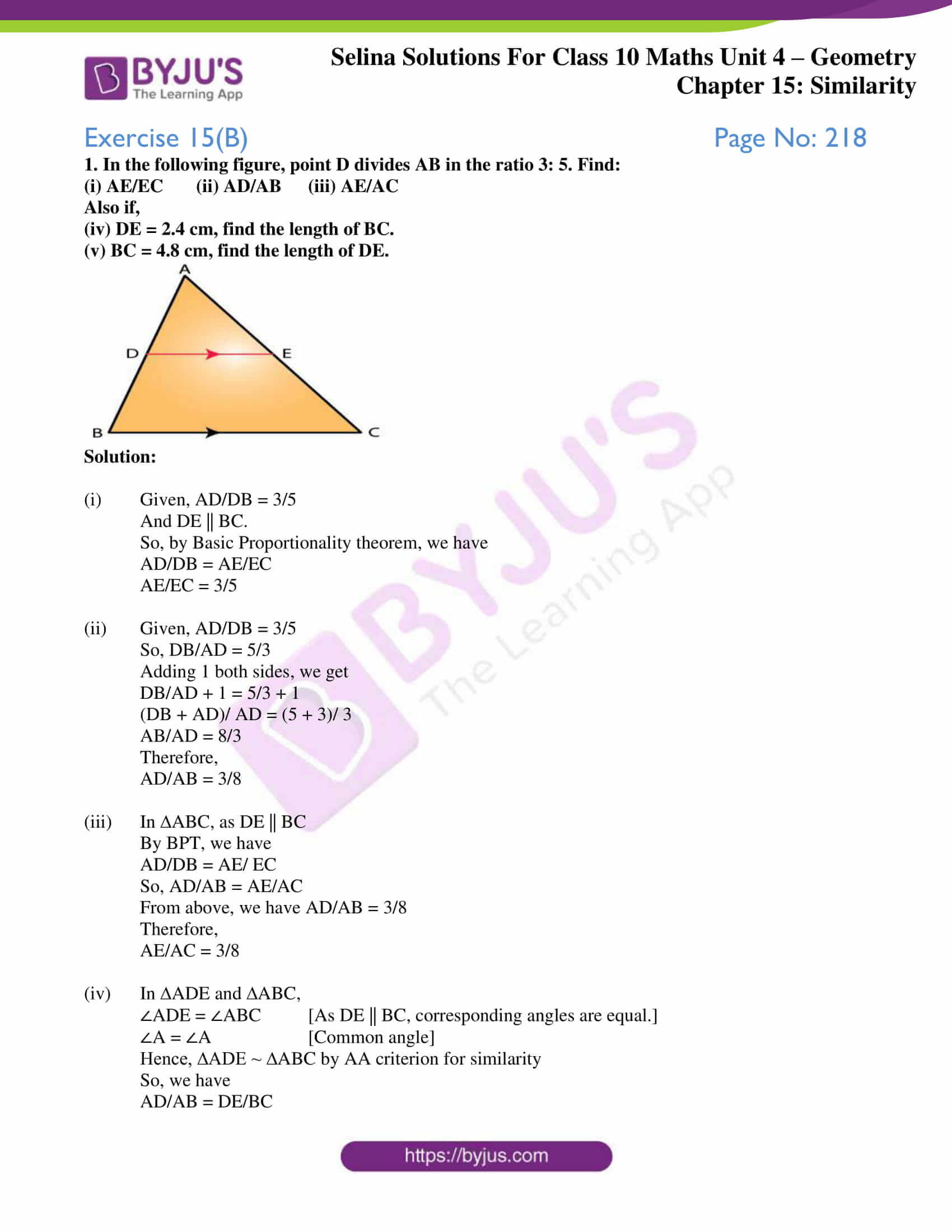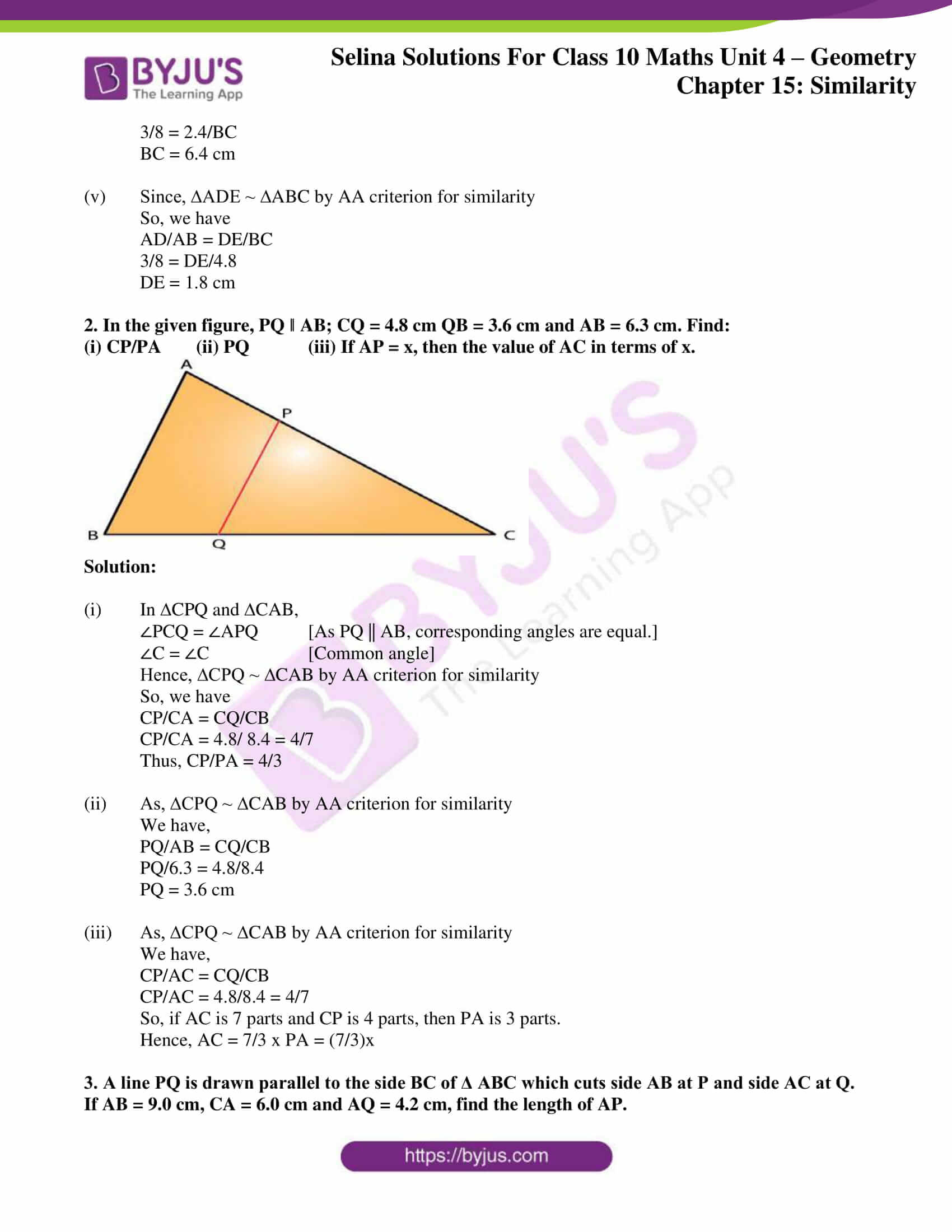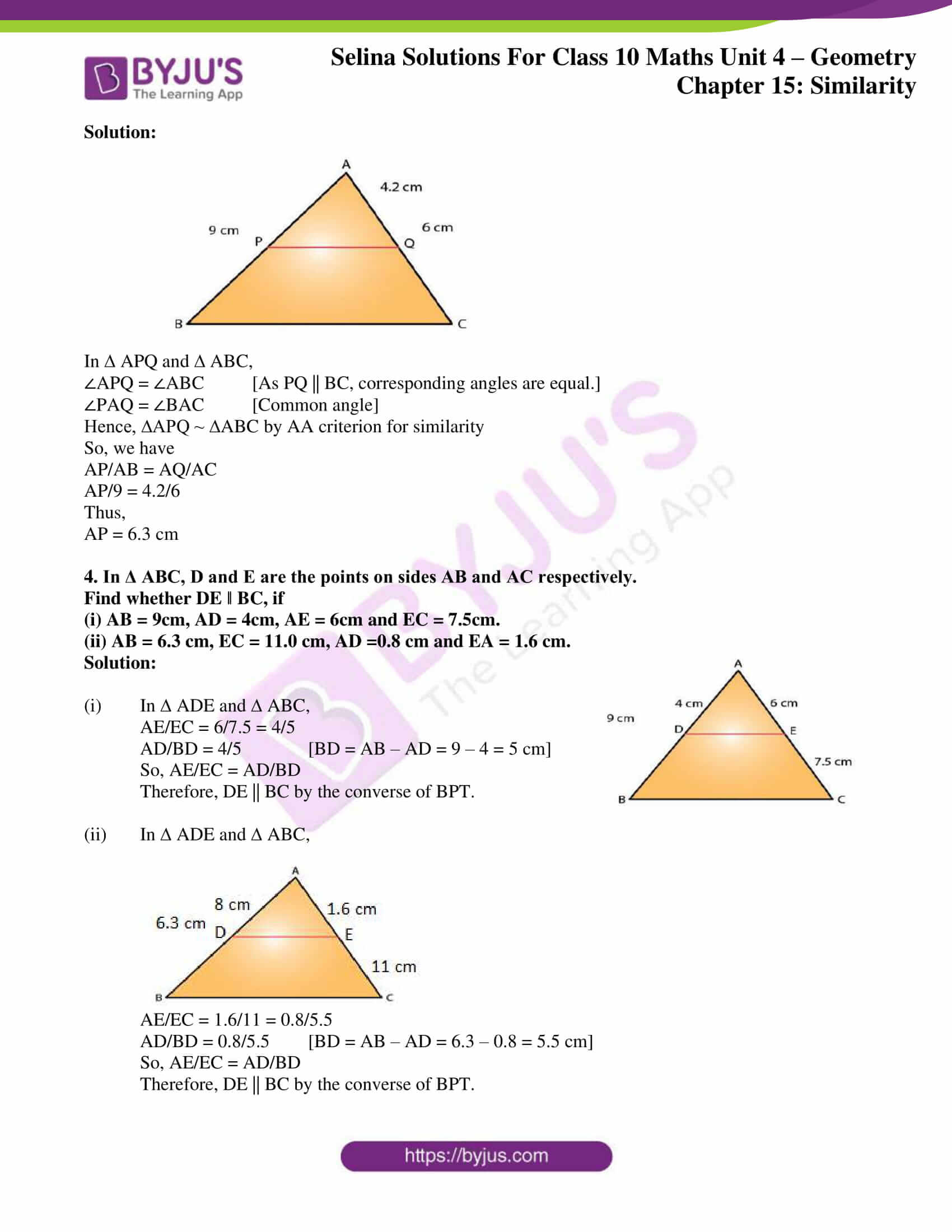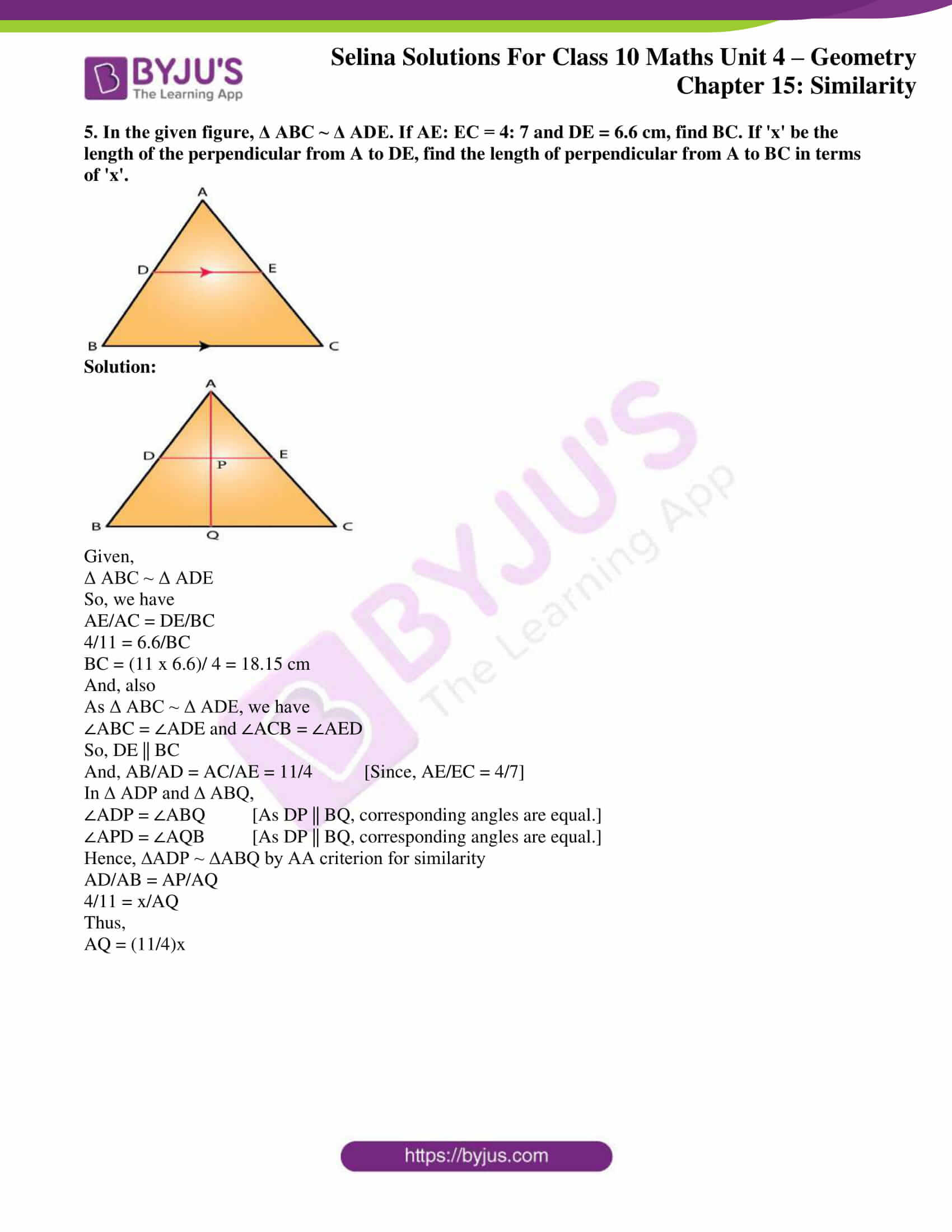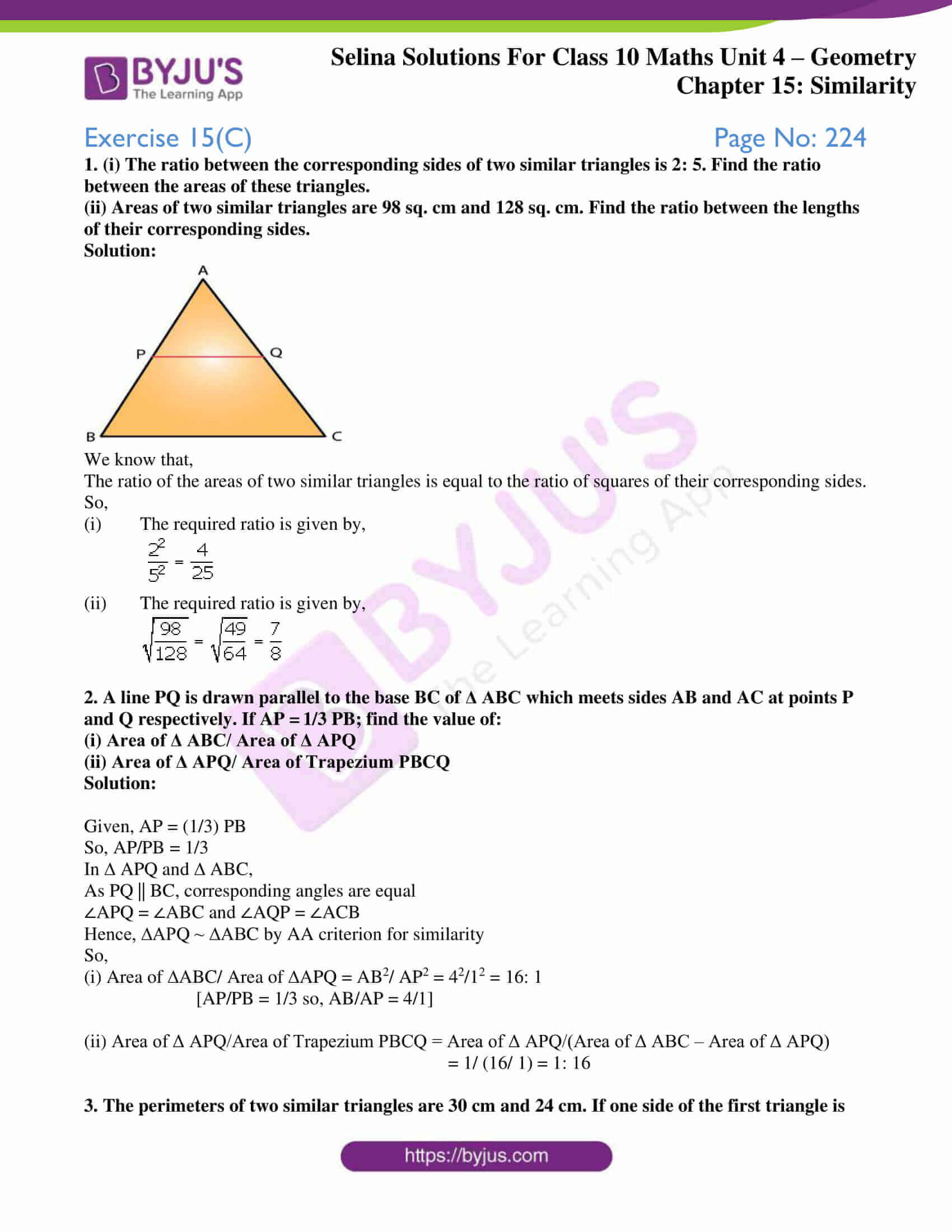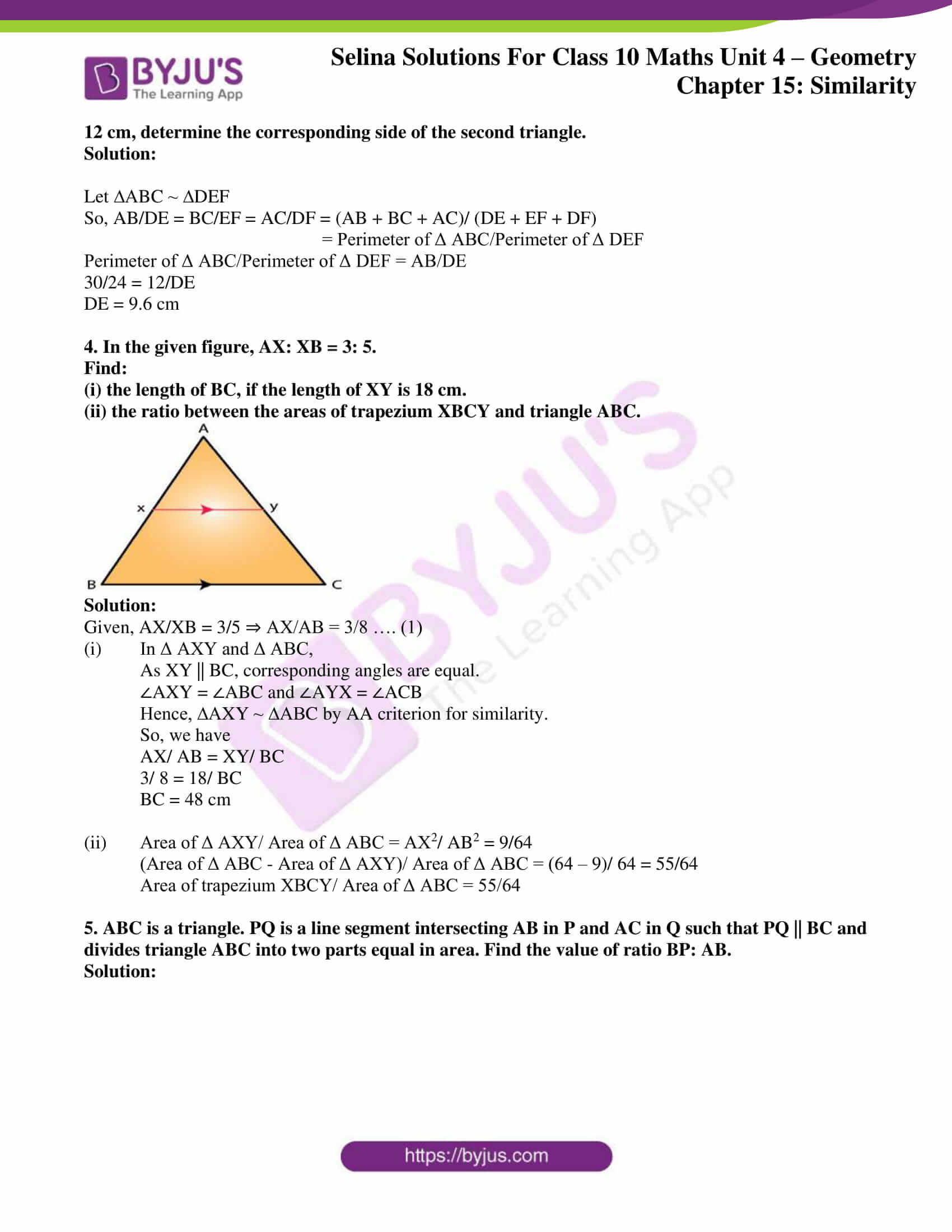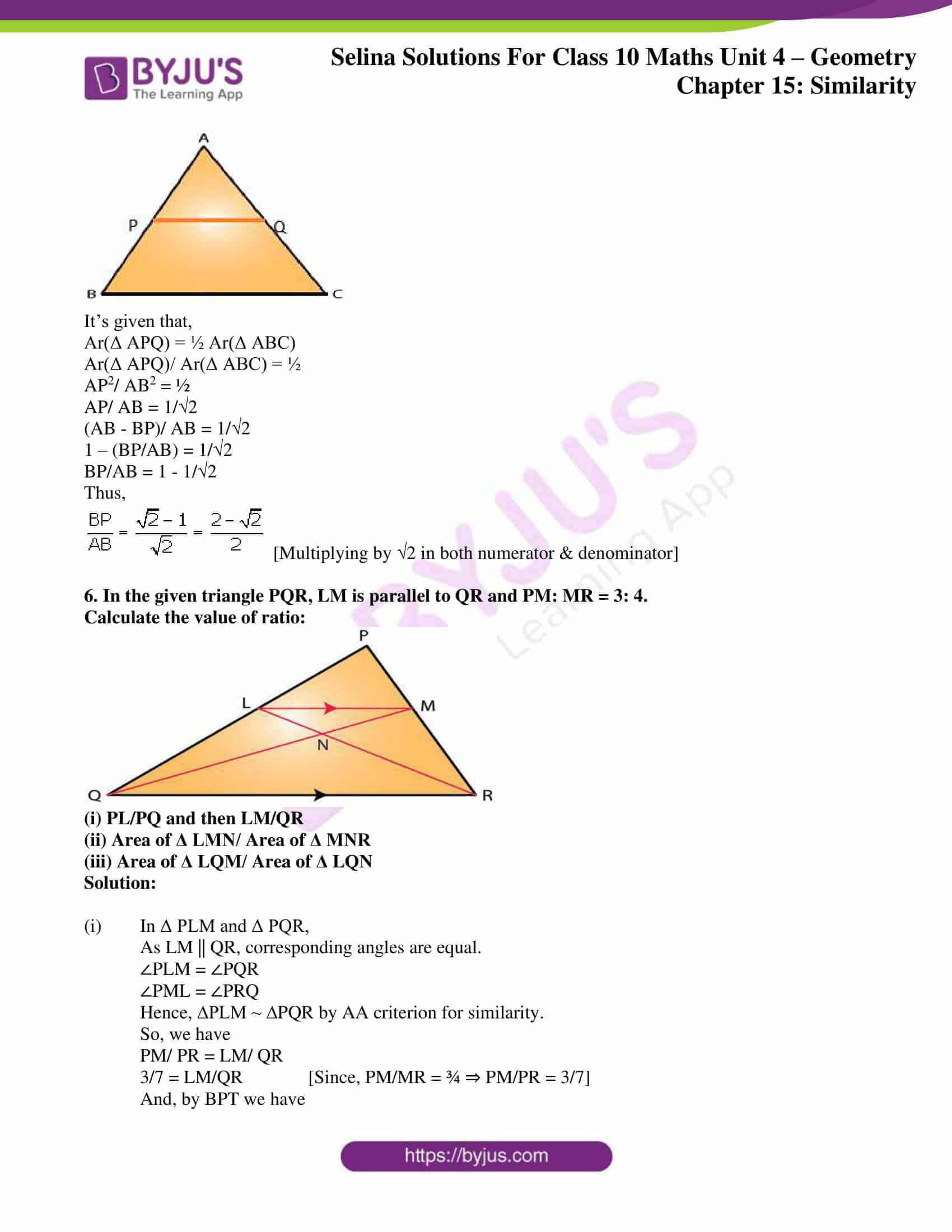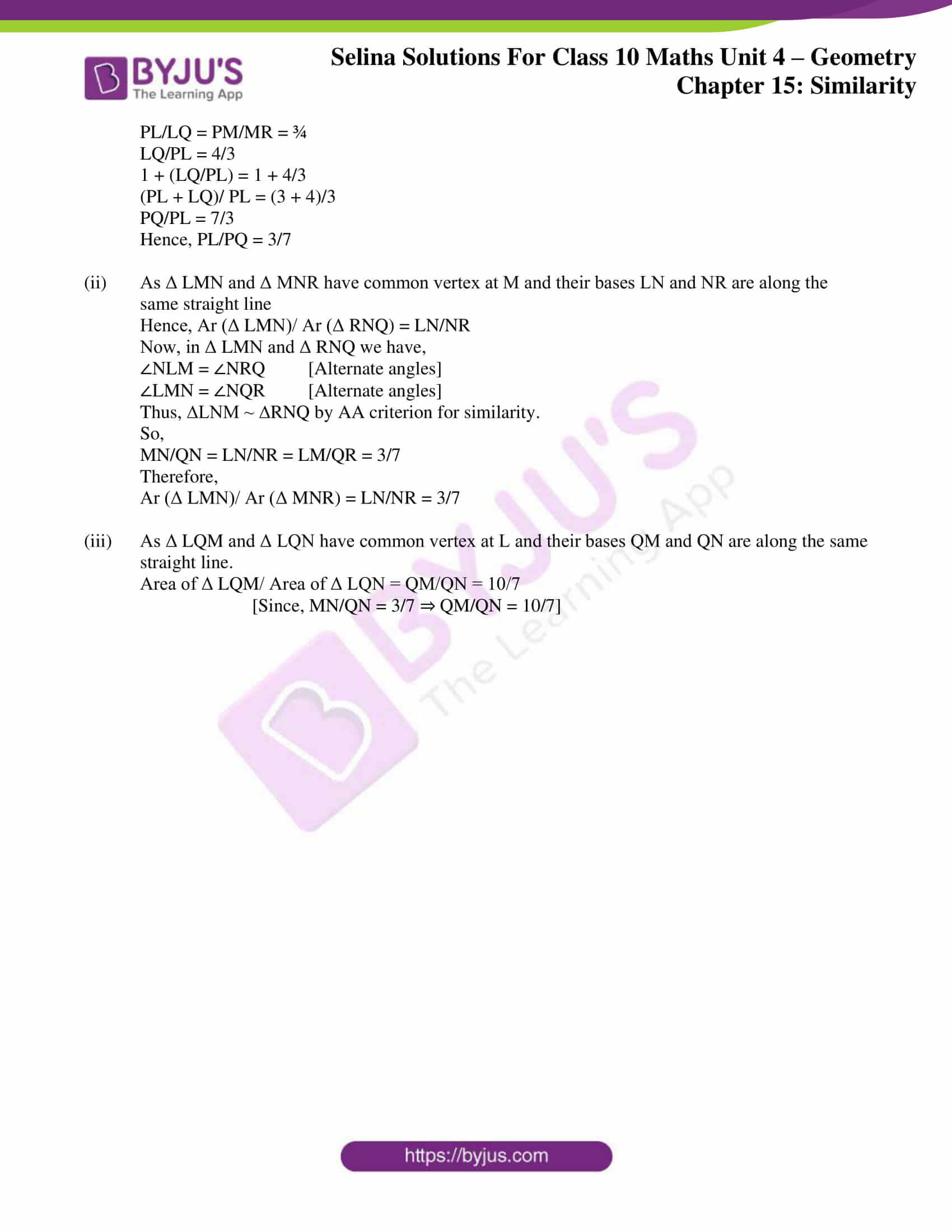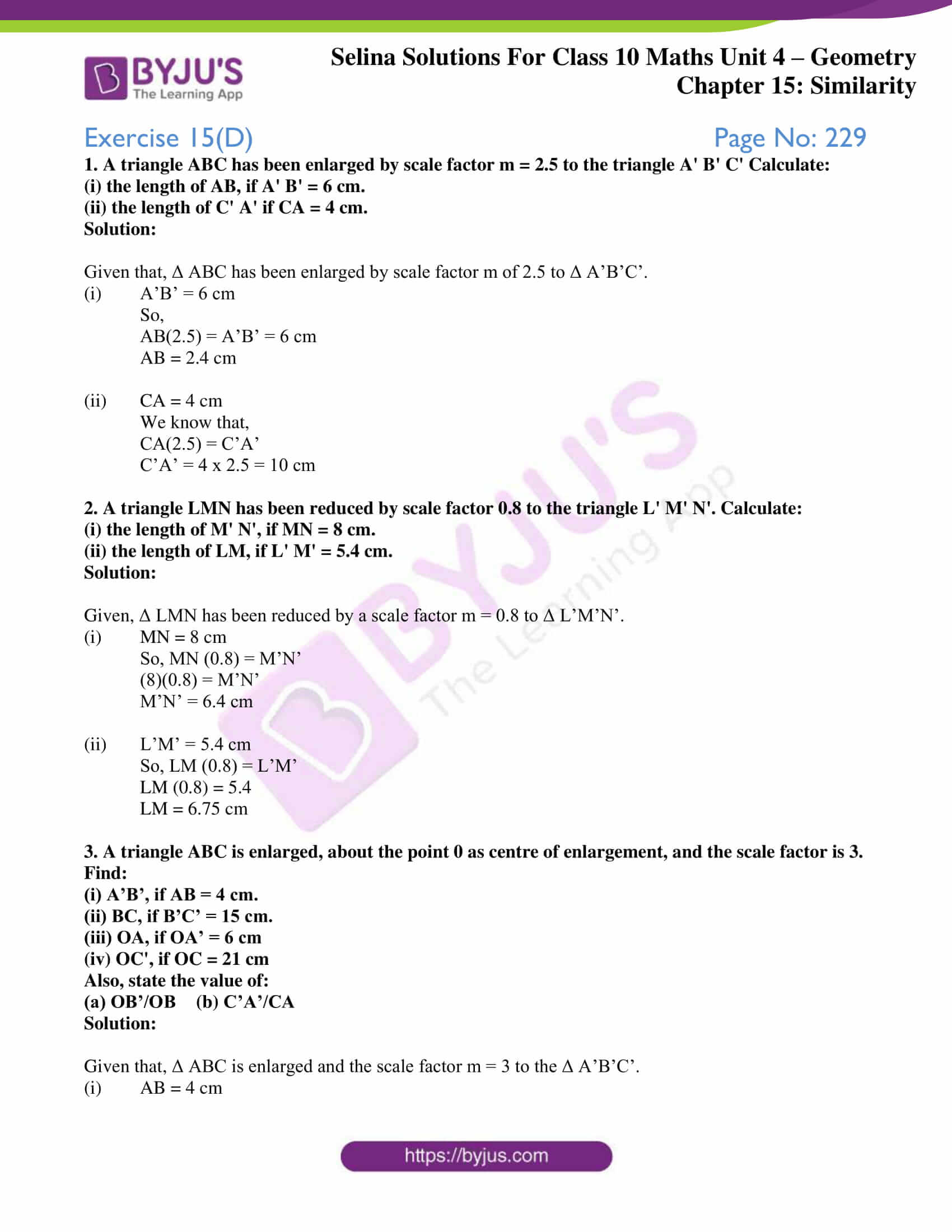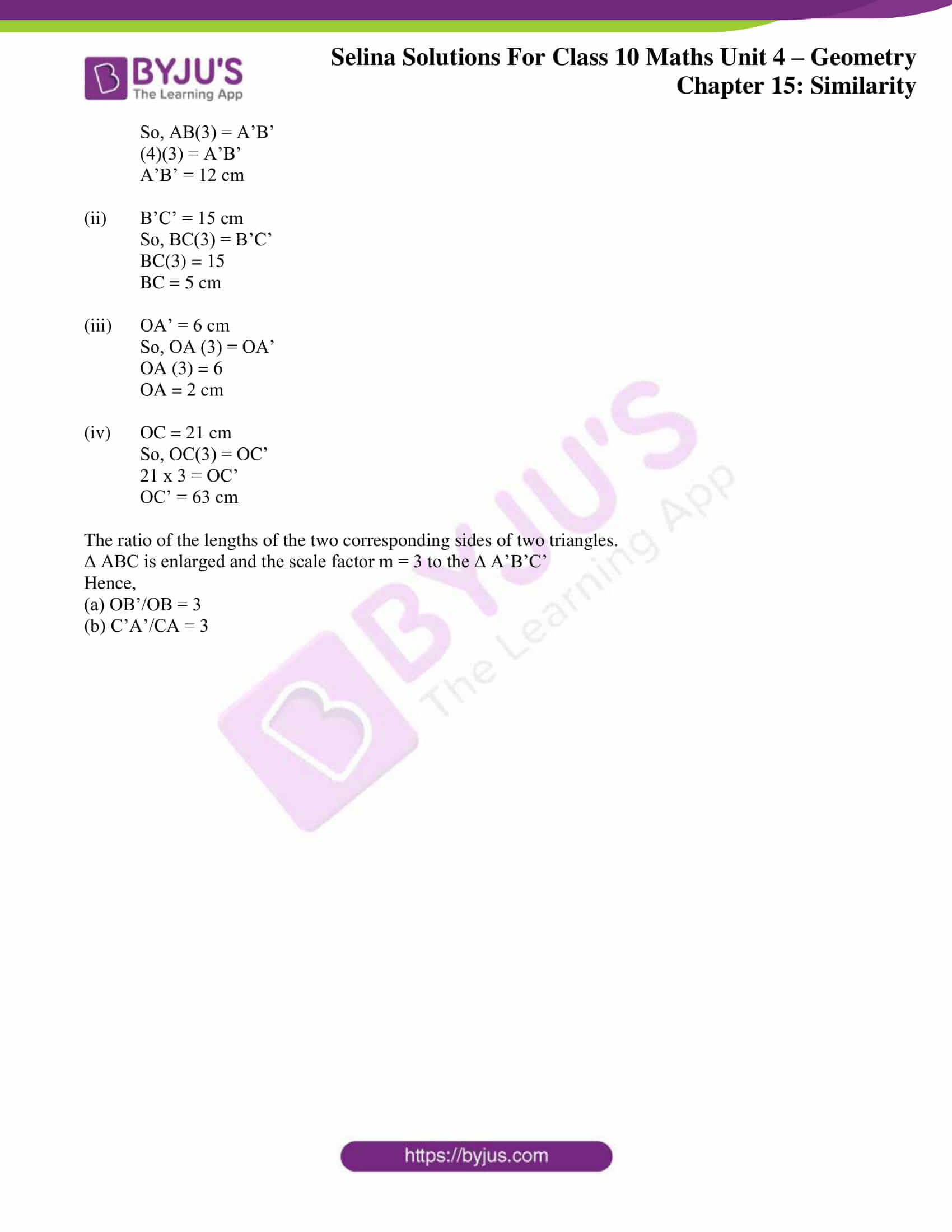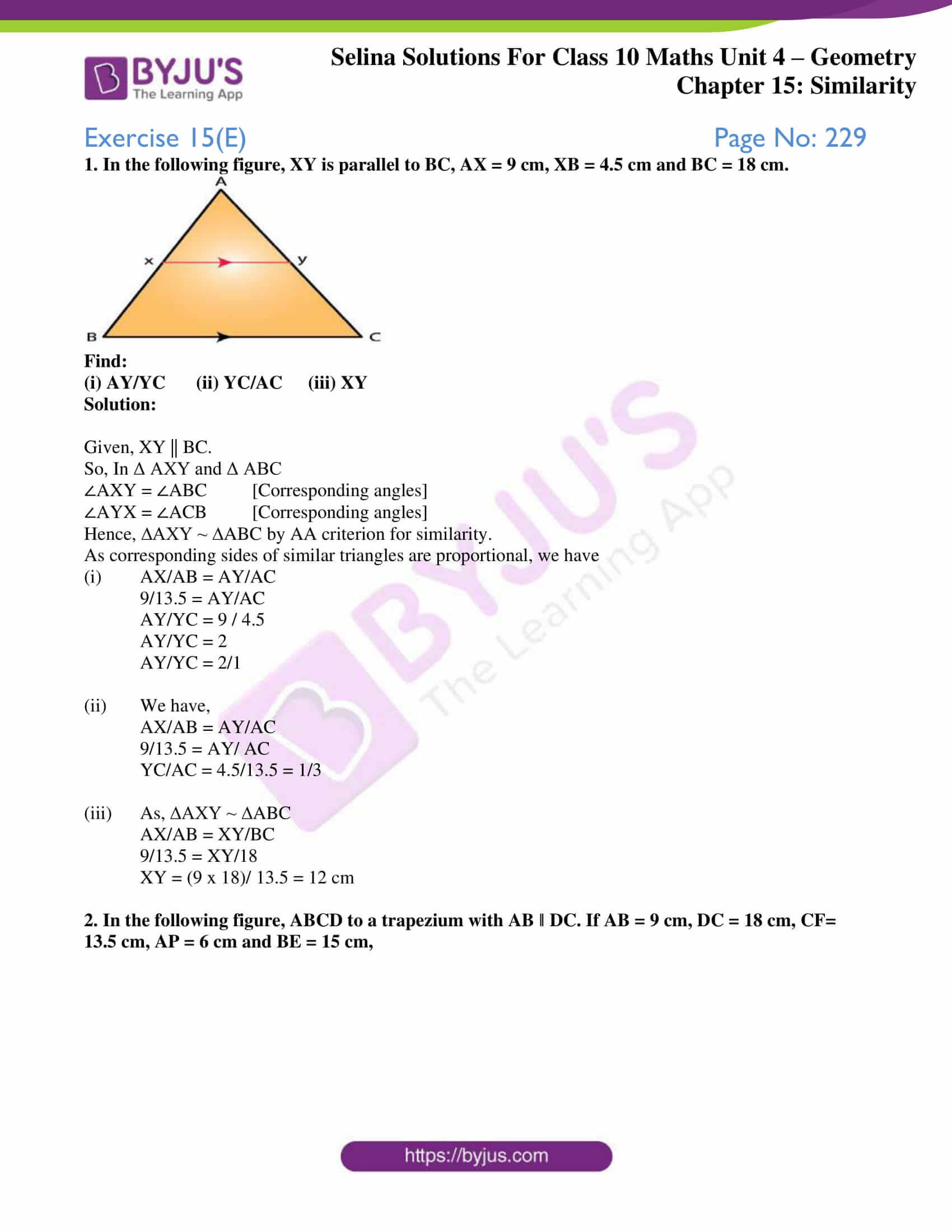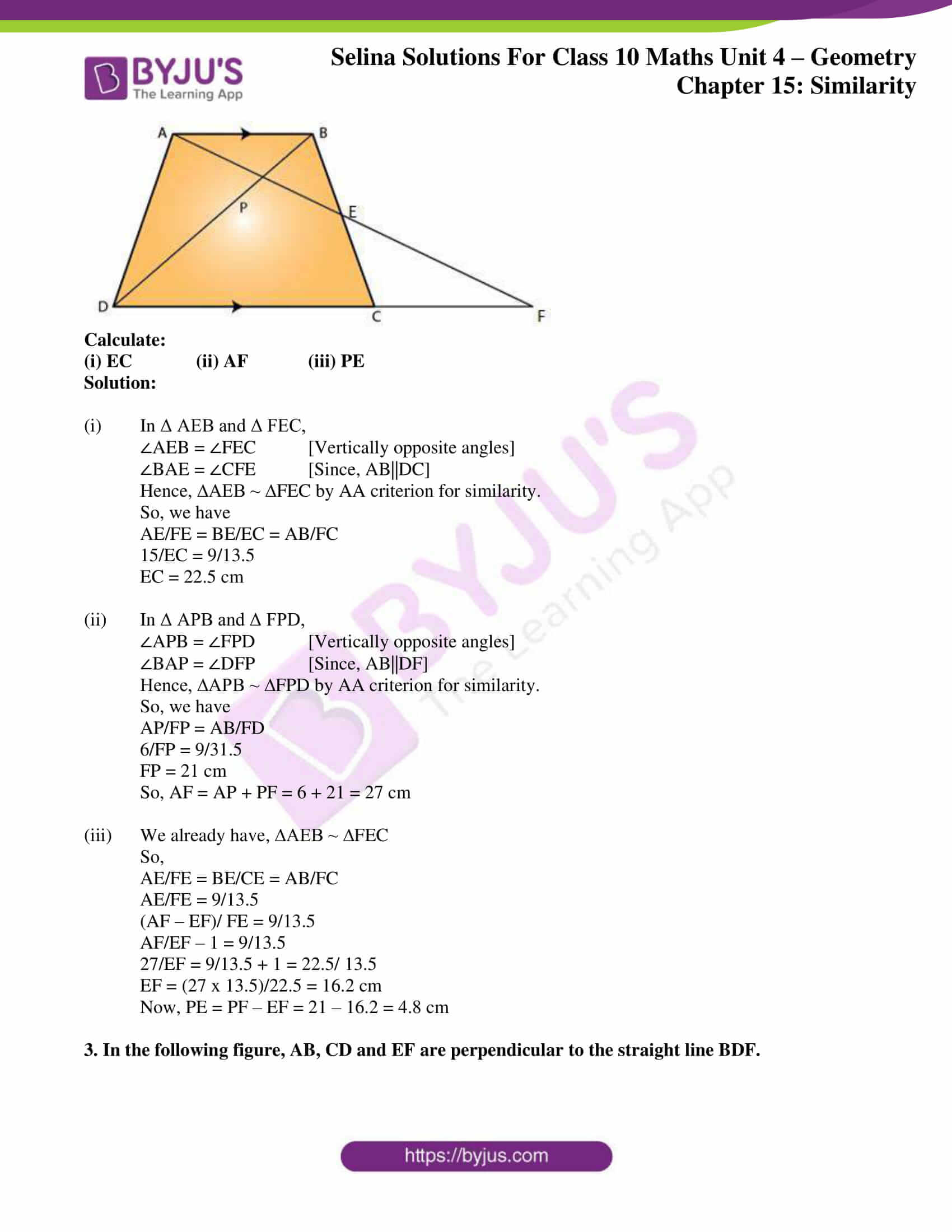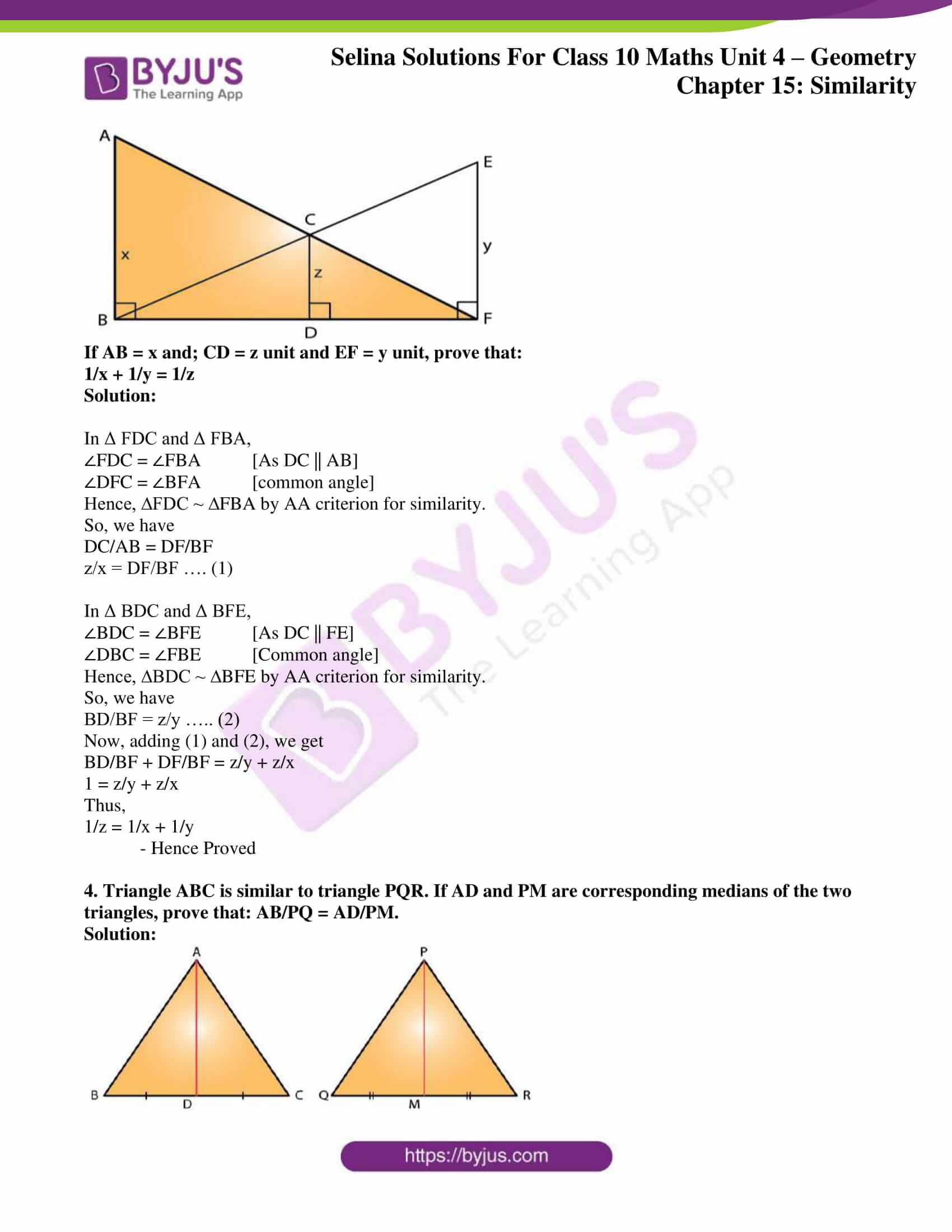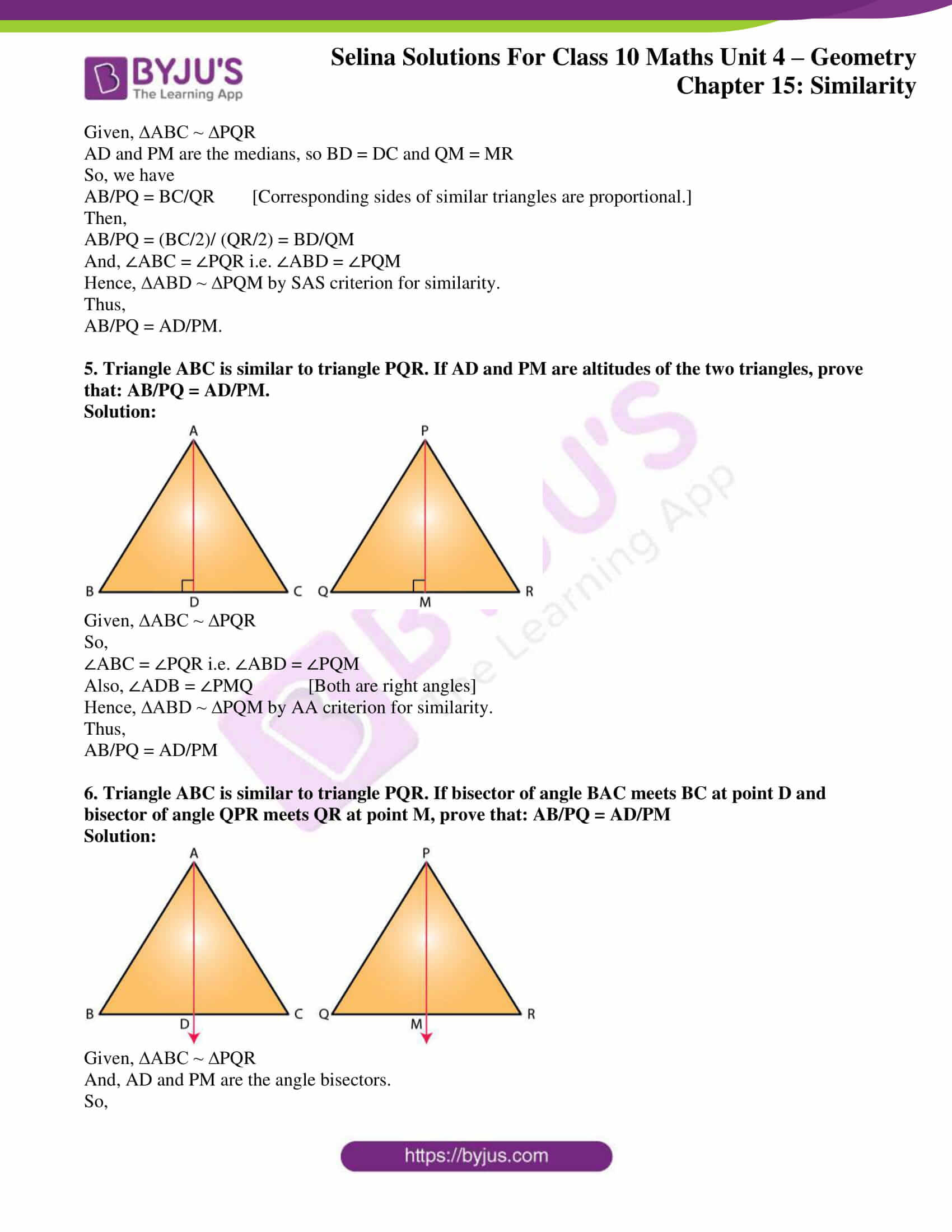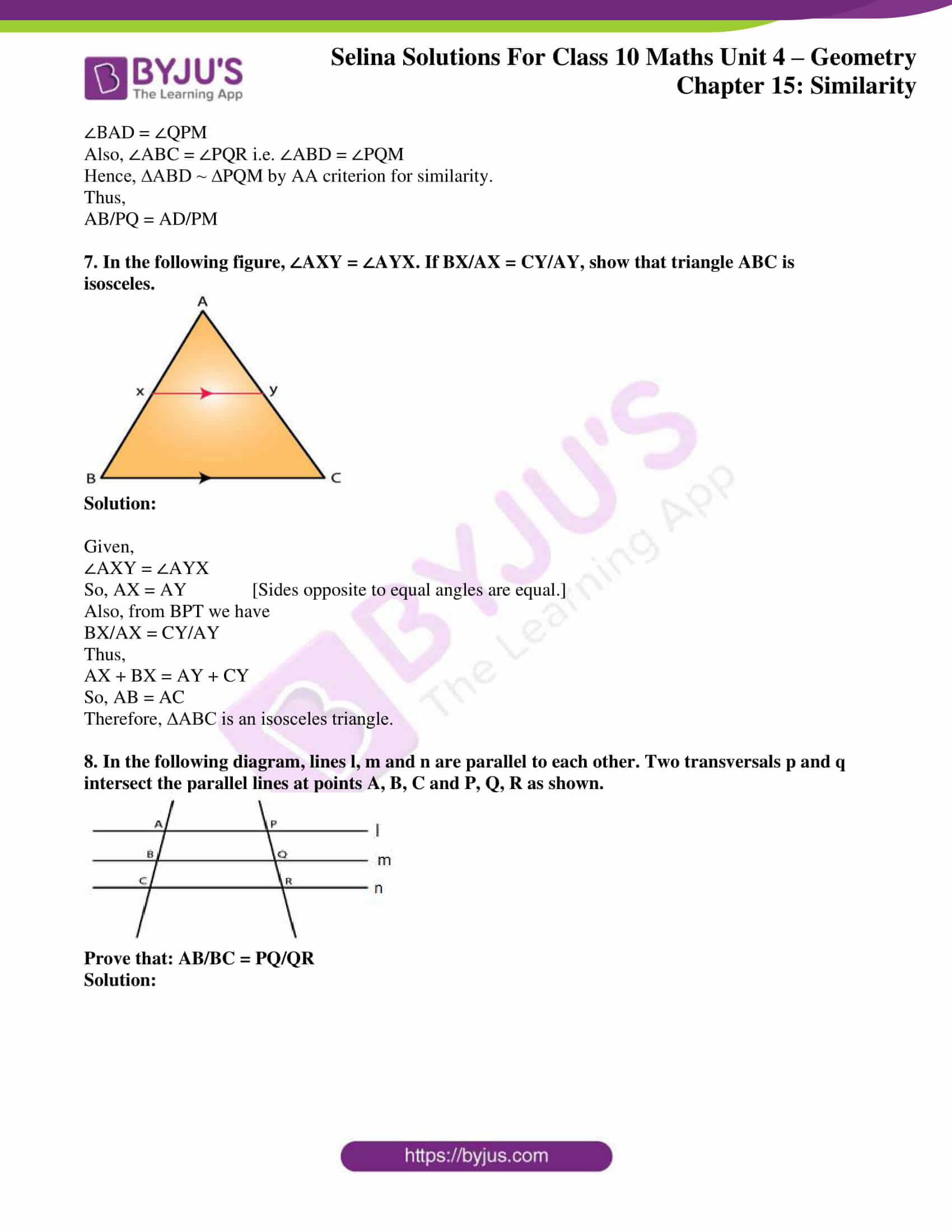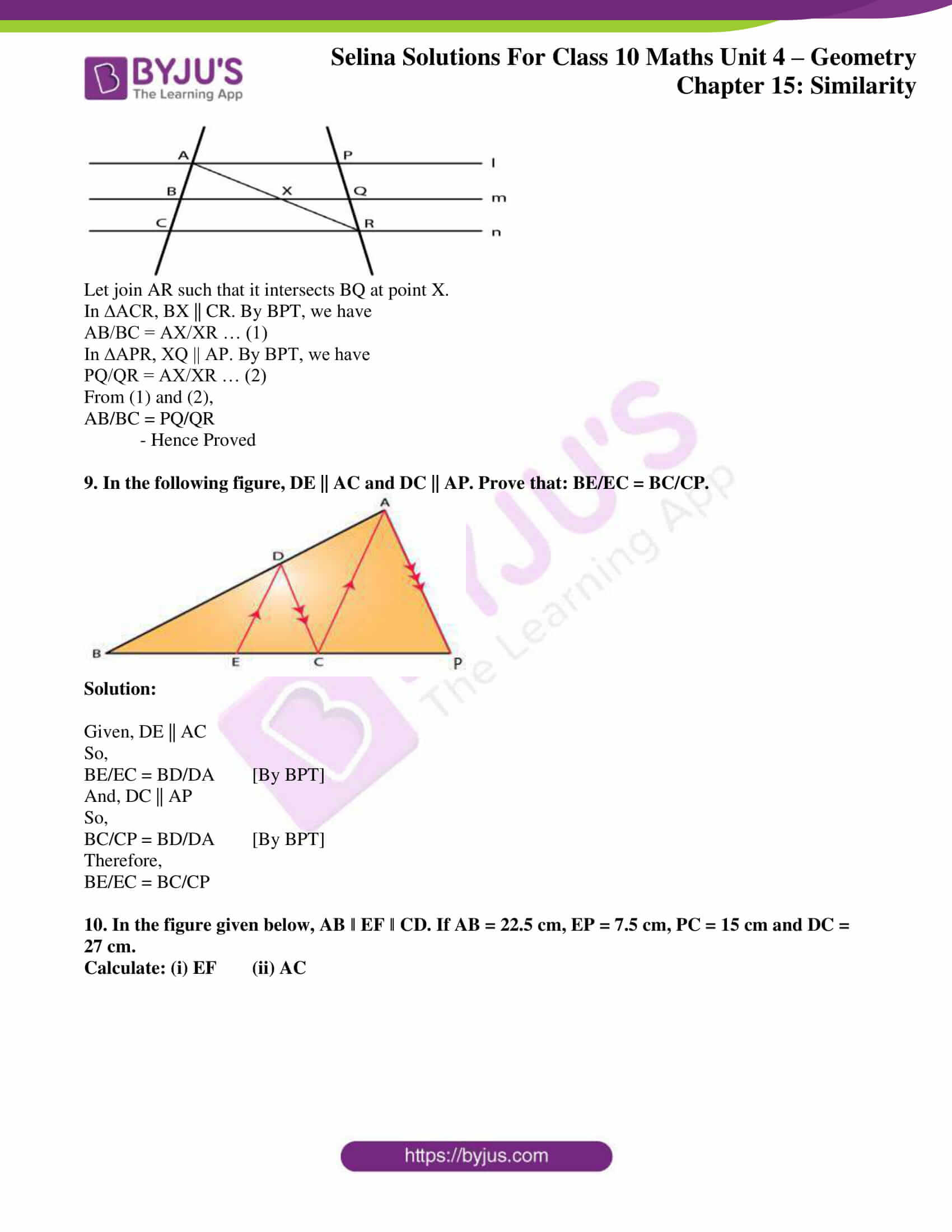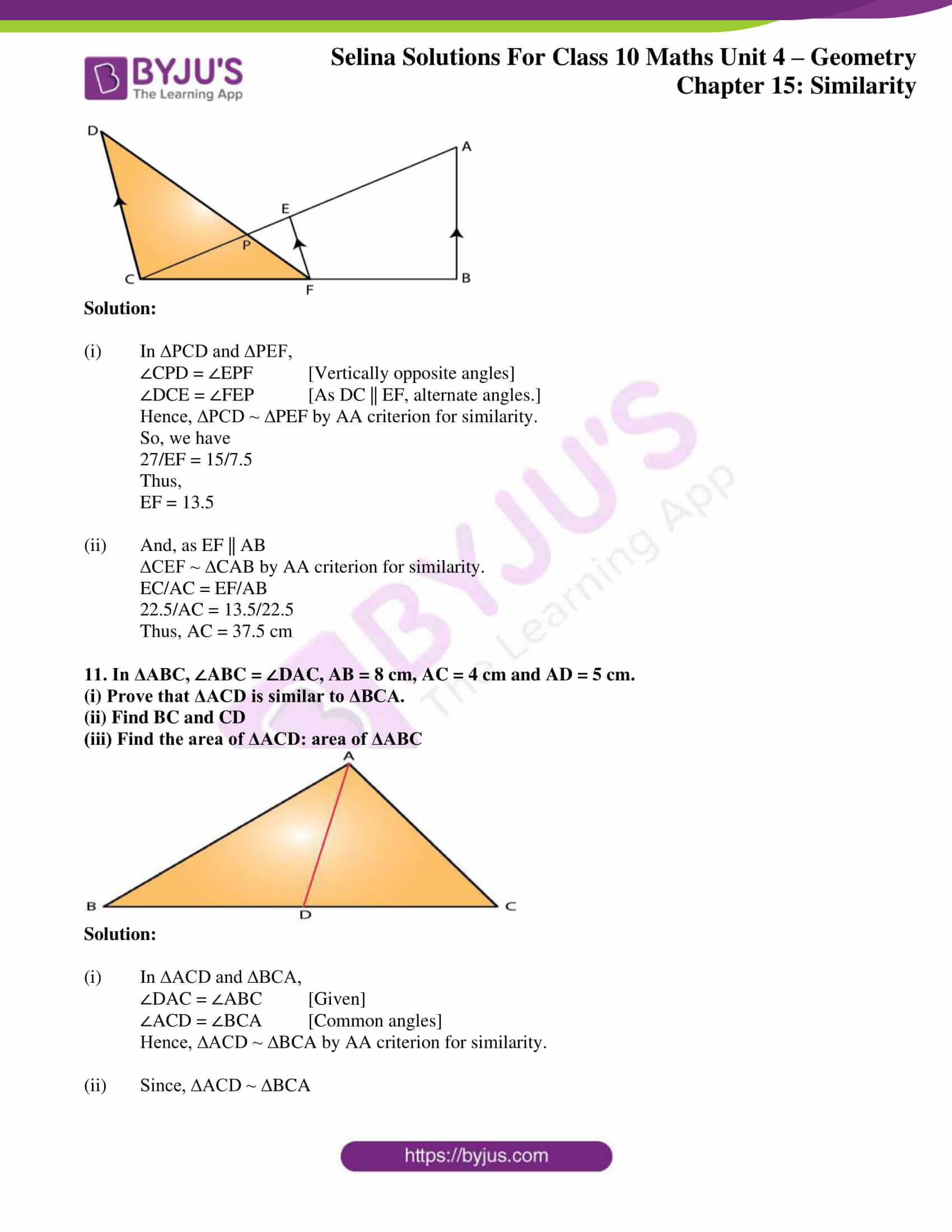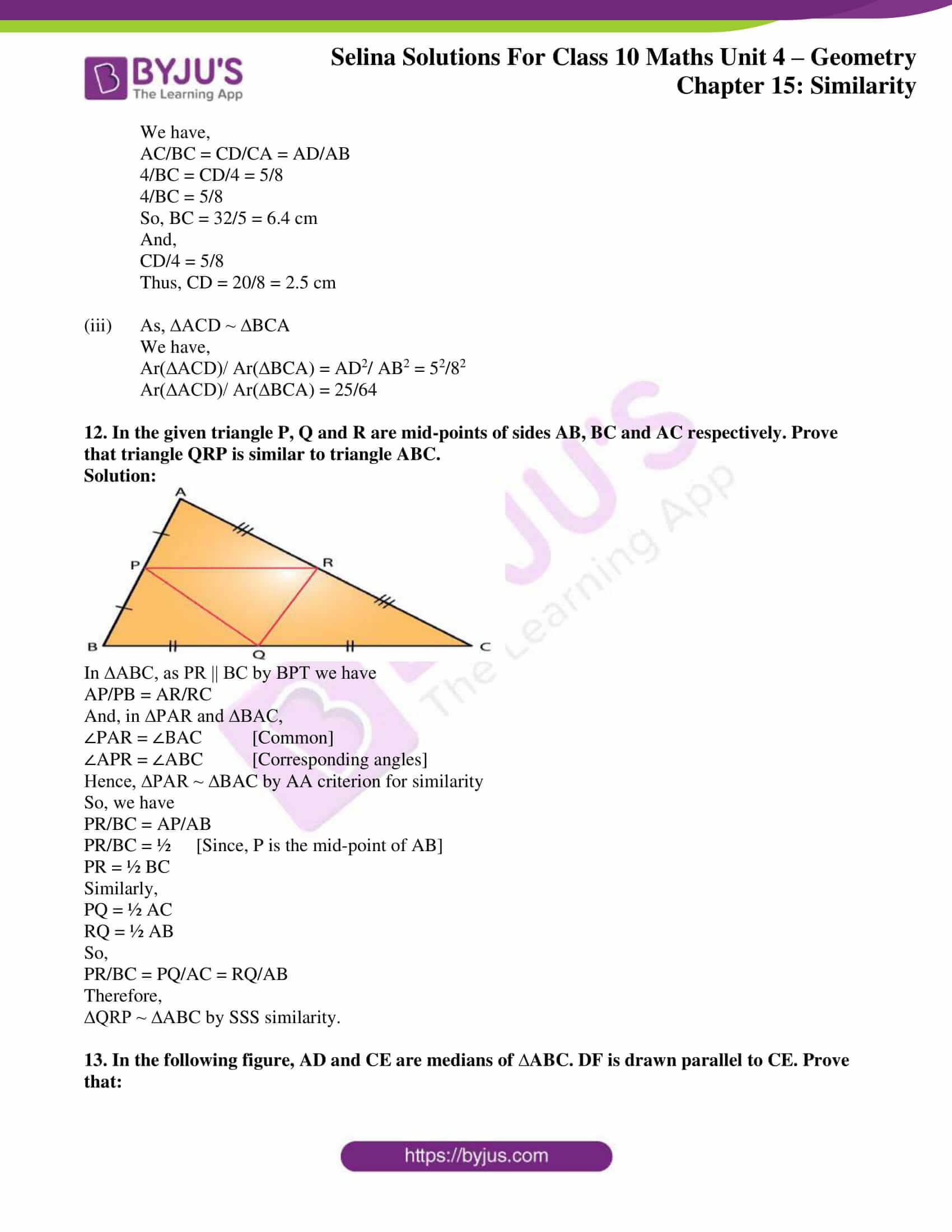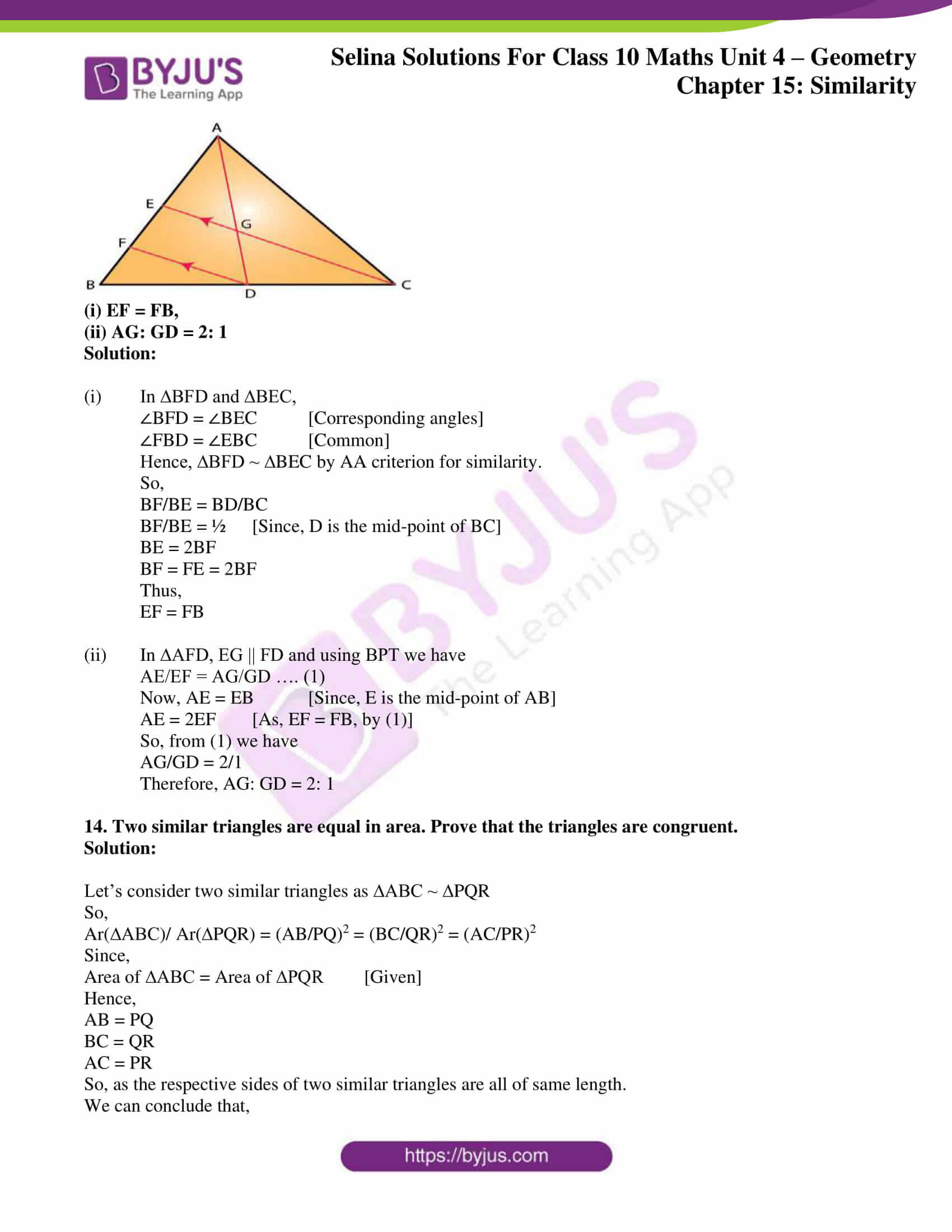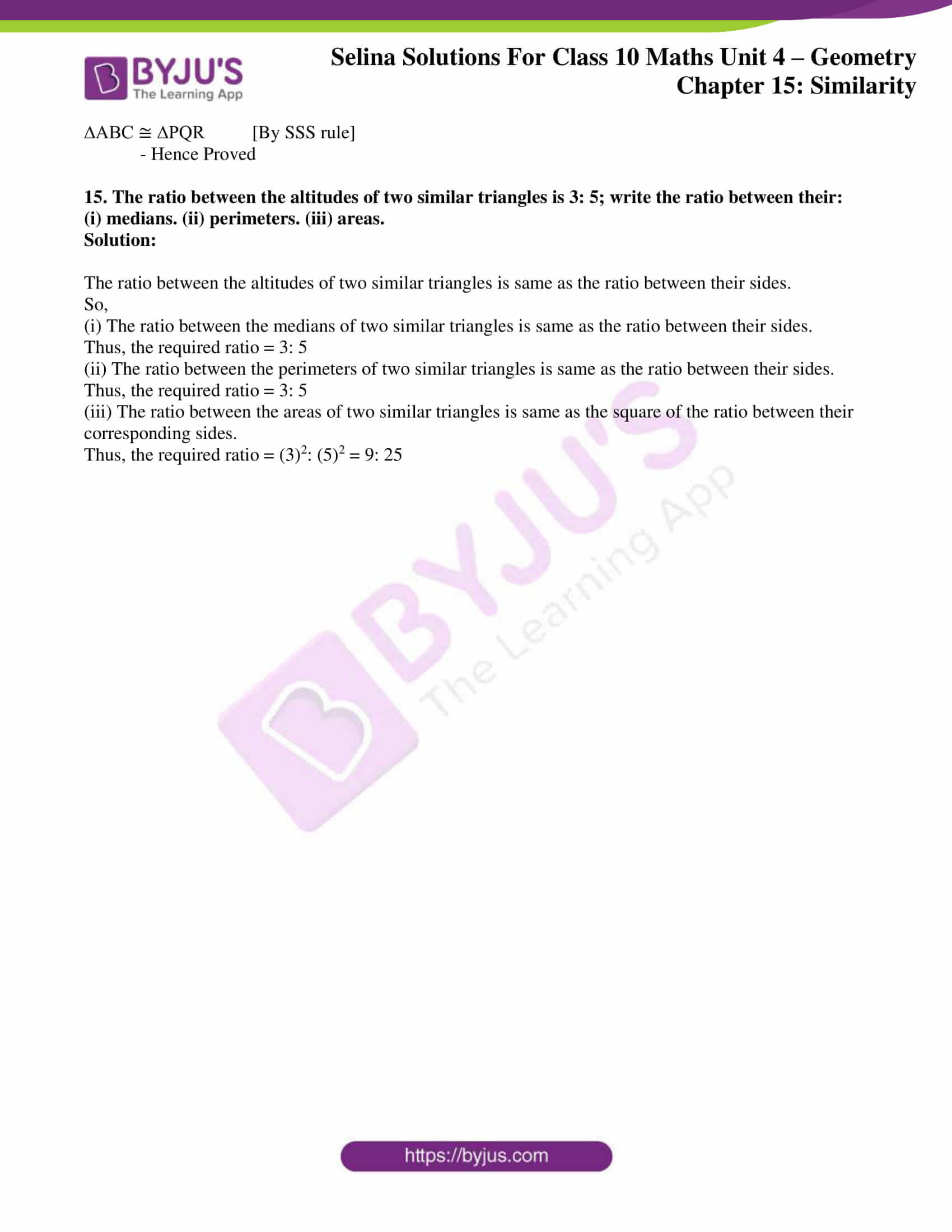## Access Selina Solutions Concise Maths Class 10 Chapter 15 Similarity (With Applications to Maps and Models)

### Exercise 15(A) Page No: 213

1. In the figure, given below, straight lines AB and CD intersect at P; and AC || BD. Prove that:

(i) ∆APC and ∆BPD are similar.

(ii) If BD = 2.4 cm, AC = 3.6 cm, PD = 4.0 cm and PB = 3.2 cm; find the lengths of PA and PC.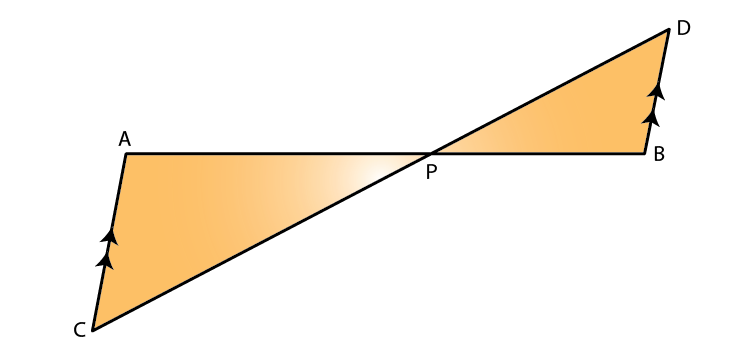Solution:

(i) In ∆APC and ∆BPD, we have

∠APC = ∠BPD [Vertically opposite angles]

∠ACP = ∠BDP [Alternate angles as, AC || BD]

Thus, ∆APC ~ ∆BPD by AA similarity criterion

(ii) So, by corresponding parts of similar triangles, we have

PA/PB = PC/PD = AC/BD

Given, BD = 2.4 cm, AC = 3.6 cm, PD = 4.0 cm and PB = 3.2 cm

PA/(3.2) = PC/4 = 3.6/2.4

PA/3.2 = 3.6/2.4 and PC/4 = 3.6/2.4

Thus,

PA = (3.6 x 3.2)/ 2.4 = 4.8 cm and

PC = (3.6 x 4)/ 2.4 = 6 cm

2. In a trapezium ABCD, side AB is parallel to side DC; and the diagonals AC and BD intersect each other at point P. Prove that:

(i) Δ APB is similar to Δ CPD.

(ii) PA x PD = PB x PC.

Solution: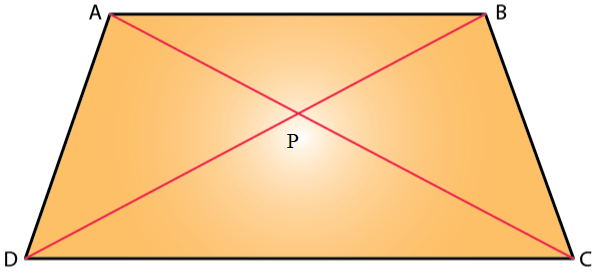(i) In ∆APB and ∆CPD, we have

∠APB = ∠CPD [Vertically opposite angles]

∠ABP = ∠CDP [Alternate angles as, AB||DC]

Thus, ∆APB ~ ∆CPD by AA similarity criterion.

(ii) As ∆APB ~ ∆CPD

Since the corresponding sides of similar triangles are proportional, we have

PA/PC = PB/PD

Thus,

PA x PD = PB x PC

3. P is a point on side BC of a parallelogram ABCD. If DP produced meets AB produced at point L, prove that:

(i) DP : PL = DC : BL.

(ii) DL : DP = AL : DC.

Solution: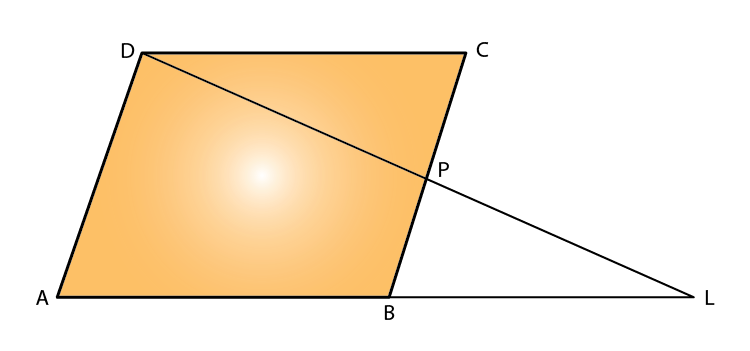So, by BPT

DP/PL = AB/BL

And, since ABCD is a parallelogram, AB = DC

Hence,

DP/PL = DC/BL

i.e., DP : PL = DC : BL

So, by BPT

DL/DP = AL/AB

And, since ABCD is a parallelogram, AB = DC

Hence,

DL/DP = AL/AB

i.e., DL : DP = AL : DC

4. In quadrilateral ABCD, the diagonals AC and BD intersect each other at point O. If AO = 2CO and BO = 2DO; show that:

(i) Δ AOB is similar to Δ COD.

(ii) OA x OD = OB x OC.

Solution: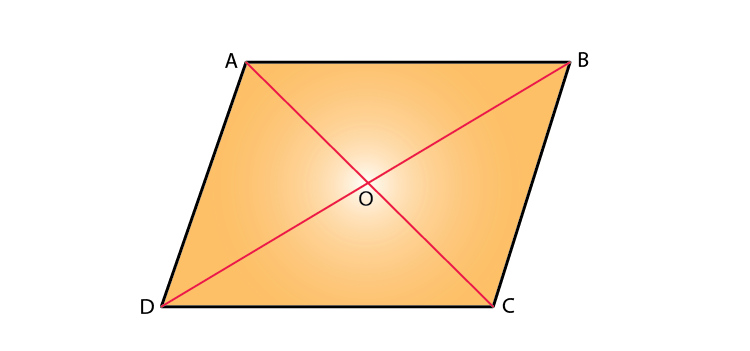(i) Given,

AO = 2CO and BO = 2DO,

AO/CO = 2/1 = BO/DO

And,

∠AOB = ∠DOC [Vertically opposite angles]

Hence, ∆AOB ~ ∆COD [SAS criterion for similarity]

(ii) As, AO/CO = 2/1 = BO/DO [Given]

Thus,

OA x OD = OB x OC

5. In Δ ABC, angle ABC is equal to twice the angle ACB, and bisector of angle ABC meets the opposite side at point P. Show that :

(i) CB : BA = CP : PA

(ii) AB x BC = BP x CA

Solution: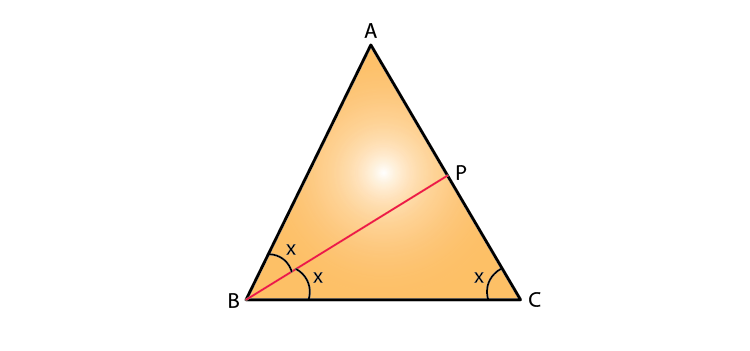(i) In Δ ABC, we have

∠ABC = 2 ∠ACB [Given]

Now, let ∠ACB = x

So, ∠ABC = 2x

Also given, BP is bisector of ∠ABC

Thus, ∠ABP = ∠PBC = x

By using the angle bisector theorem,

i.e. the bisector of an angle divides the side opposite to it in the ratio of other two sides.

Therefore, CB: BA = CP: PA.

(ii) In Δ ABC and Δ APB,

∠ABC = ∠APB [Exterior angle property]

∠BCP = ∠ABP [Given]

Thus, ∆ABC ~ ∆APB by AA criterion for similarity

Now, since corresponding sides of similar triangles are proportional we have

CA/AB = BC/BP

Therefore, AB x BC = BP x CA

6. In Δ ABC, BM ⊥ AC and CN ⊥ AB; show that: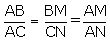Solution: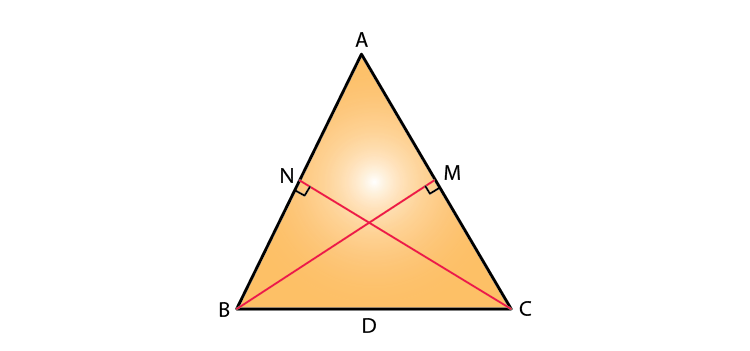In Δ ABM and Δ ACN,

∠AMB = ∠ANC [Since, BM ⊥ AC and CN ⊥ AB]

∠BAM = ∠CAN [Common angle]

Hence, ∆ABM ~ ∆ACN by AA criterion for similarity

So, by corresponding sides of similar triangles we have7. In the given figure, DE ‖ BC, AE = 15 cm, EC = 9 cm, NC = 6 cm and BN = 24 cm.

(i) Write all possible pairs of similar triangles.

(ii) Find the lengths of ME and DM.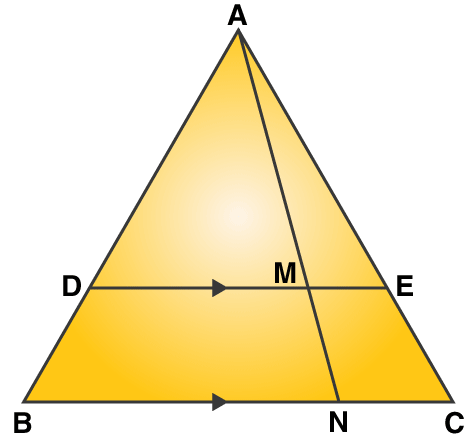Solution:

(i) In Δ AME and Δ ANC,

∠AME = ∠ANC [Since DE || BC so, ME || NC]

∠MAE = ∠NAC [Common angle]

Hence, ∆AME ~ ∆ANC by AA criterion for similarity

In Δ ADM and Δ ABN,

∠ADM = ∠ABN [Since DE || BC so, DM || BN]

∠DAM = ∠BAN [Common angle]

Hence, ∆ADM ~ ∆ABN by AA criterion for similarity

In Δ ADE and Δ ABC,

∠ADE = ∠ABC [Since DE || BC so, ME || NC]

∠AED = ∠ACB [Since DE || BC]

Hence, ∆ADE ~ ∆ABC by AA criterion for similarity

(ii) Proved above that, ∆AME ~ ∆ANC

So as corresponding sides of similar triangles are proportional, we have

ME/NC = AE/AC

ME/ 6 = 15/ 24

ME = 3.75 cm

And, ∆ADE ~ ∆ABC [Proved above]

So as corresponding sides of similar triangles are proportional, we have

AD/AB = AE/AC = 15/24 …. (1)

Also, ∆ADM ~ ∆ABN [Proved above]

So as corresponding sides of similar triangles are proportional, we have

DM/BN = AD/AB = 15/24 …. From (1)

DM/ 24 = 15/ 24

DM = 15 cm

8. In the given figure, AD = AE and AD2 = BD x EC. Prove that: triangles ABD and CAE are similar.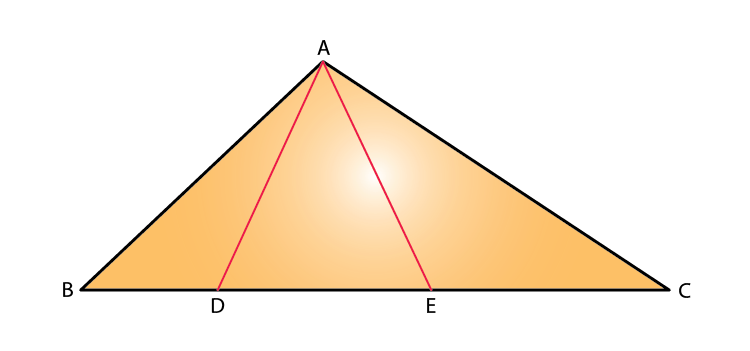Solution:

In Δ ABD and Δ CAE,

∠ADE = ∠AED [Angles opposite to equal sides are equal.]

And, AD2 = BD x EC [Given]

Thus, ∆ABD ~ ∆CAE by SAS criterion for similarity.

9. In the given figure, AB ‖ DC, BO = 6 cm and DQ = 8 cm; find: BP x DO.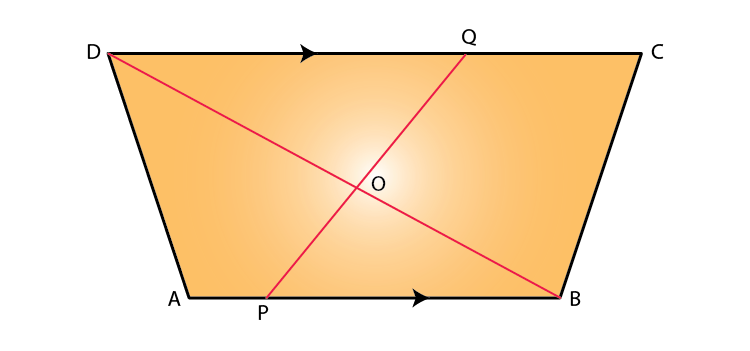Solution:

In Δ DOQ and Δ BOP,

∠QDO = ∠PBO [As AB || DC so, PB || DQ.]

So, ∠DOQ = ∠BOP [Vertically opposite angles]

Hence, ∆DOQ ~ ∆BOP by AA criterion for similarity

Since, corresponding sides of similar triangles are proportional we have

DO/BO = DQ/BP

DO/6 = 8/BP

BP x DO = 48 cm2

10. Angle BAC of triangle ABC is obtuse and AB = AC. P is a point in BC such that PC = 12 cm. PQ and PR are perpendiculars to sides AB and AC respectively. If PQ = 15 cm and PR = 9 cm; find the length of PB.

Solution: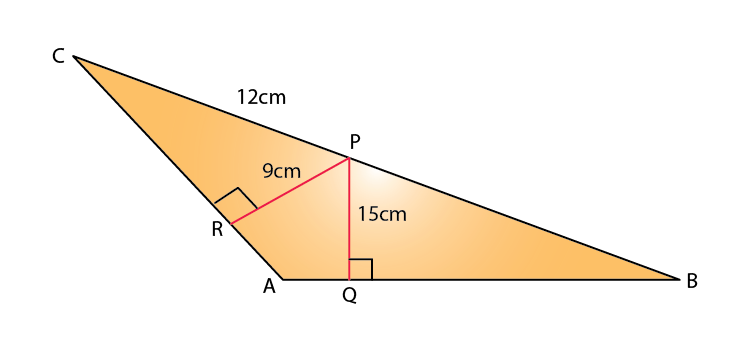In Δ ABC,

AC = AB [Given]

So, ∠ABC = ∠ACB [Angles opposite to equal sides are equal.]

In Δ PRC and Δ PQB,

∠ABC = ∠ACB

∠PRC = ∠PQB [Both are right angles.]

Hence, ∆PRC ~ ∆PQB by AA criterion for similarity

Since, corresponding sides of similar triangles are proportional we have

PR/PQ = RC/QB = PC/PB

PR/PQ = PC/PB

9/15 = 12/PB

Thus,

PB = 20 cm

11. State, true or false:

(i) Two similar polygons are necessarily congruent.

(ii) Two congruent polygons are necessarily similar.

(iii) All equiangular triangles are similar.

(iv) All isosceles triangles are similar.

(v) Two isosceles-right triangles are similar.

(vi) Two isosceles triangles are similar, if an angle of one is congruent to the corresponding angle of the other.

(vii) The diagonals of a trapezium, divide each other into proportional segments.

Solution:

(i) False

(ii) True

(iii) True

(iv) False

(v) True

(vi) True

(vii) True

12. Given: GHE = DFE = 90o, DH = 8, DF = 12, DG = 3x – 1 and DE = 4x + 2.

Find: the lengths of segments DG and DE.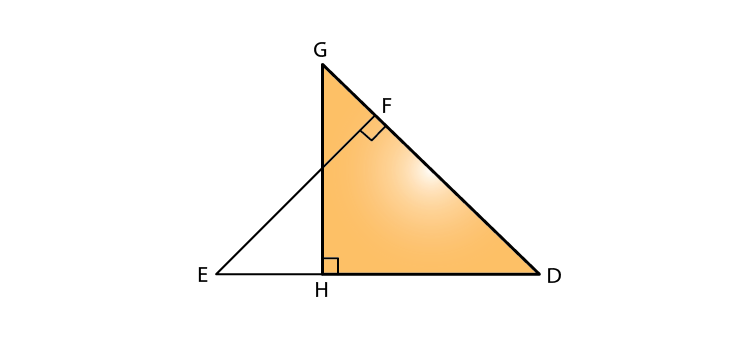Solution:

In Δ DHG and Δ DFE,

∠GHD = ∠DFE = 90o

∠D = ∠D [Common]

Thus, ∆DHG ~ ∆DFE by AA criterion for similarity

So, we have

DH/DF = DG/DE

8/12 = (3x – 1)/ (4x + 2)

32x + 16 = 36x – 12

28 = 4x

x = 7

Hence,

DG = 3 x 7 – 1 = 20

DE = 4 x 7 + 2 = 30

13. D is a point on the side BC of triangle ABC such that angle ADC is equal to angle BAC. Prove that: CA2 = CB x CD.

Solution: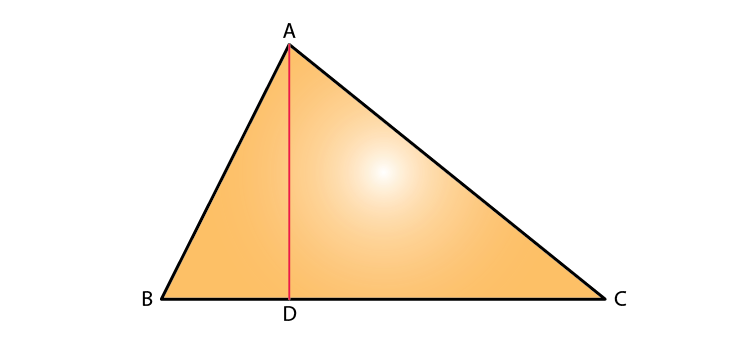In Δ ADC and Δ BAC,

∠ACD = ∠ACB [Common]

Thus, ∆ADC ~ ∆BAC by AA criterion for similarity

So, we have

CA/CB = CD/CA

Therefore,

CA2 = CB x CD

14. In the given figure, ∆ ABC and ∆ AMP are right angled at B and M respectively.

Given AC = 10 cm, AP = 15 cm and PM = 12 cm.

(i) ∆ ABC ~ ∆ AMP.

(ii) Find AB and BC.

Solution: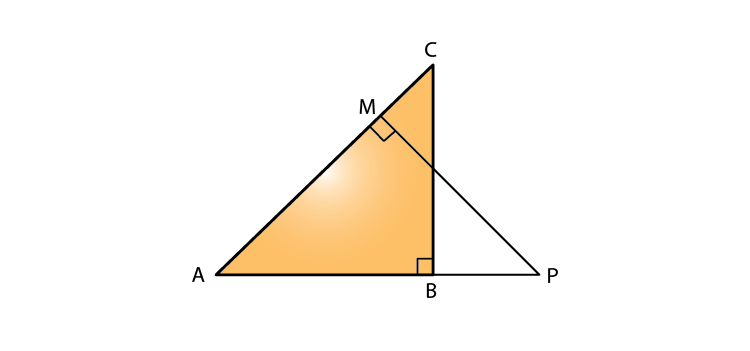(i) In ∆ ABC and ∆ AMP, we have

∠BAC = ∠PAM [Common]

∠ABC = ∠PMA [Each = 90o]

Hence, ∆ABC ~ ∆AMP by AA criterion for similarity

(ii) Now, in right triangle AMP

By using Pythagoras theorem, we have

AM = √(AP2 – PM2) = √(152 – 122) = 9

As ∆ABC ~ ∆AMP,

AB/AM = BC/PM = AC/AP

AB/9 = BC/12 = 10/15

So,

AB/9 = 10/15

AB = (10 x 9)/ 15 = 6 cm

BC/12 = 10/ 15

BC = 8 cm

### Exercise 15(B) Page No: 218

1. In the following figure, point D divides AB in the ratio 3: 5. Find:

(i) AE/EC (ii) AD/AB (iii) AE/AC

Also if,

(iv) DE = 2.4 cm, find the length of BC.

(v) BC = 4.8 cm, find the length of DE.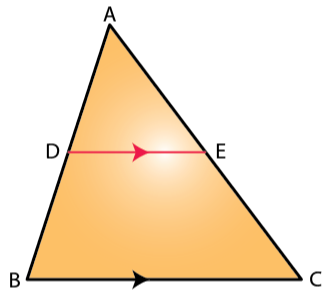Solution:

And DE || BC.

So, by Basic Proportionality theorem, we have

AE/EC = 3/5

Adding 1 both sides, we get

DB/AD + 1 = 5/3 + 1

Therefore,

(iii) In ∆ABC, as DE || BC

By BPT, we have

From above, we have AD/AB = 3/8

Therefore,

AE/AC = 3/8

∠ADE = ∠ABC [As DE || BC, corresponding angles are equal.]

∠A = ∠A [Common angle]

Hence, ∆ADE ~ ∆ABC by AA criterion for similarity

So, we have

3/8 = 2.4/BC

BC = 6.4 cm

(v) Since, ∆ADE ~ ∆ABC by AA criterion for similarity

So, we have

3/8 = DE/4.8

DE = 1.8 cm

2. In the given figure, PQ ‖ AB; CQ = 4.8 cm QB = 3.6 cm and AB = 6.3 cm. Find:

(i) CP/PA (ii) PQ (iii) If AP = x, then the value of AC in terms of x.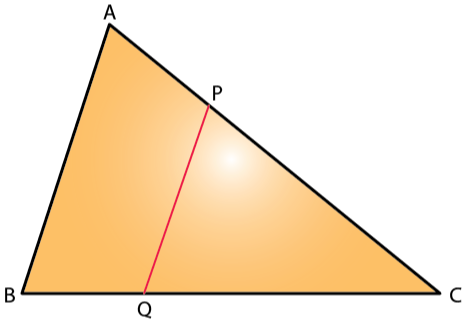Solution:

(i) In ∆CPQ and ∆CAB,

∠PCQ = ∠APQ [As PQ || AB, corresponding angles are equal.]

∠C = ∠C [Common angle]

Hence, ∆CPQ ~ ∆CAB by AA criterion for similarity

So, we have

CP/CA = CQ/CB

CP/CA = 4.8/ 8.4 = 4/7

Thus, CP/PA = 4/3

(ii) As, ∆CPQ ~ ∆CAB by AA criterion for similarity

We have,

PQ/AB = CQ/CB

PQ/6.3 = 4.8/8.4

PQ = 3.6 cm

(iii) As, ∆CPQ ~ ∆CAB by AA criterion for similarity

We have,

CP/AC = CQ/CB

CP/AC = 4.8/8.4 = 4/7

So, if AC is 7 parts and CP is 4 parts, then PA is 3 parts.

Hence, AC = 7/3 x PA = (7/3)x

3. A line PQ is drawn parallel to the side BC of Δ ABC which cuts side AB at P and side AC at Q. If AB = 9.0 cm, CA = 6.0 cm and AQ = 4.2 cm, find the length of AP.

Solution: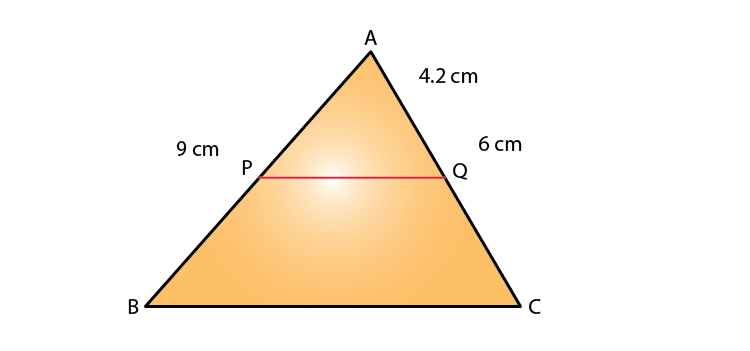In ∆ APQ and ∆ ABC,

∠APQ = ∠ABC [As PQ || BC, corresponding angles are equal.]

∠PAQ = ∠BAC [Common angle]

Hence, ∆APQ ~ ∆ABC by AA criterion for similarity

So, we have

AP/AB = AQ/AC

AP/9 = 4.2/6

Thus,

AP = 6.3 cm

4. In Δ ABC, D and E are the points on sides AB and AC respectively.

Find whether DE ‖ BC, if

(i) AB = 9cm, AD = 4cm, AE = 6cm and EC = 7.5cm.

(ii) AB = 6.3 cm, EC = 11.0 cm, AD =0.8 cm and EA = 1.6 cm.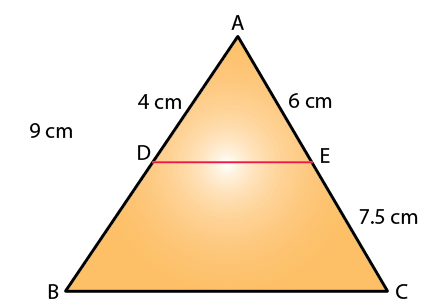Solution:

(i) In ∆ ADE and ∆ ABC,

AE/EC = 6/7.5 = 4/5

AD/BD = 4/5 [BD = AB – AD = 9 – 4 = 5 cm]

Therefore, DE || BC by the converse of BPT.

(ii) In ∆ ADE and ∆ ABC,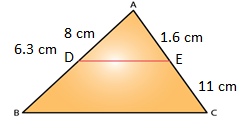AE/EC = 1.6/11 = 0.8/5.5

AD/BD = 0.8/5.5 [BD = AB – AD = 6.3 – 0.8 = 5.5 cm]

Therefore, DE || BC by the converse of BPT.

5. In the given figure, Δ ABC ~ Δ ADE. If AE: EC = 4: 7 and DE = 6.6 cm, find BC. If ‘x’ be the length of the perpendicular from A to DE, find the length of perpendicular from A to BC in terms of ‘x’.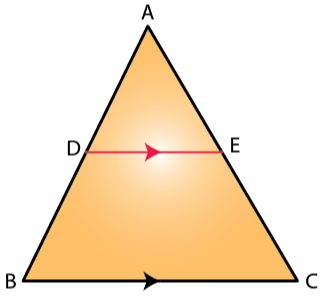Solution: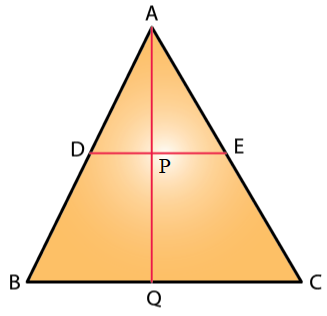Given,

So, we have

AE/AC = DE/BC

4/11 = 6.6/BC

BC = (11 x 6.6)/ 4 = 18.15 cm

And, also

As Δ ABC ~ Δ ADE, we have

∠ABC = ∠ADE and ∠ACB = ∠AED

So, DE || BC

And, AB/AD = AC/AE = 11/4 [Since, AE/EC = 4/7]

In ∆ ADP and ∆ ABQ,

∠ADP = ∠ABQ [As DP || BQ, corresponding angles are equal.]

∠APD = ∠AQB [As DP || BQ, corresponding angles are equal.]

Hence, ∆ADP ~ ∆ABQ by AA criterion for similarity

4/11 = x/AQ

Thus,

AQ = (11/4)x

### Exercise 15(C) Page No: 224

1. (i) The ratio between the corresponding sides of two similar triangles is 2: 5. Find the ratio between the areas of these triangles.

(ii) Areas of two similar triangles are 98 sq. cm and 128 sq. cm. Find the ratio between the lengths of their corresponding sides.

Solution: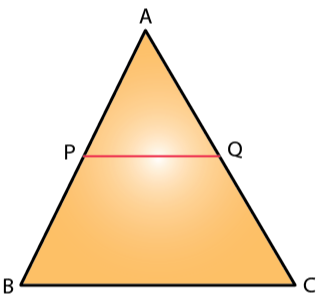We know that,

The ratio of the areas of two similar triangles is equal to the ratio of squares of their corresponding sides.

So,

(i) The required ratio is given by,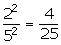(ii) The required ratio is given by,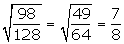2. A line PQ is drawn parallel to the base BC of Δ ABC which meets sides AB and AC at points P and Q respectively. If AP = 1/3 PB; find the value of:

(i) Area of Δ ABC/ Area of Δ APQ

(ii) Area of Δ APQ/ Area of Trapezium PBCQ

Solution:

Given, AP = (1/3) PB

So, AP/PB = 1/3

In ∆ APQ and ∆ ABC,

As PQ || BC, corresponding angles are equal

∠APQ = ∠ABC and ∠AQP = ∠ACB

Hence, ∆APQ ~ ∆ABC by AA criterion for similarity

So,

(i) Area of ∆ABC/ Area of ∆APQ = AB2/ AP2 = 42/12 = 16: 1

[AP/PB = 1/3 so, AB/AP = 4/1]

(ii) Area of Δ APQ/Area of Trapezium PBCQ = Area of Δ APQ/(Area of Δ ABC – Area of Δ APQ)

= 1/ (16/ 1) = 1: 16

3. The perimeters of two similar triangles are 30 cm and 24 cm. If one side of the first triangle is 12 cm, determine the corresponding side of the second triangle.

Solution:

Let ∆ABC ~ ∆DEF

So, AB/DE = BC/EF = AC/DF = (AB + BC + AC)/ (DE + EF + DF)

= Perimeter of Δ ABC/Perimeter of Δ DEF

Perimeter of Δ ABC/Perimeter of Δ DEF = AB/DE

30/24 = 12/DE

DE = 9.6 cm

4. In the given figure, AX: XB = 3: 5.

Find:

(i) the length of BC, if the length of XY is 18 cm.

(ii) the ratio between the areas of trapezium XBCY and triangle ABC.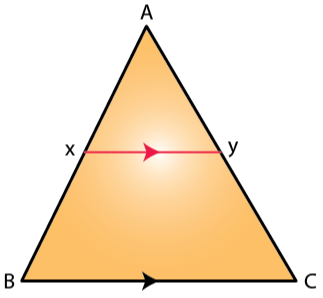Solution:

Given, AX/XB = 3/5 ⇒ AX/AB = 3/8 …. (1)

(i) In Δ AXY and Δ ABC,

As XY || BC, corresponding angles are equal.

∠AXY = ∠ABC and ∠AYX = ∠ACB

Hence, ∆AXY ~ ∆ABC by AA criterion for similarity.

So, we have

AX/ AB = XY/ BC

3/ 8 = 18/ BC

BC = 48 cm

(ii) Area of Δ AXY/ Area of Δ ABC = AX2/ AB2 = 9/64

(Area of Δ ABC – Area of Δ AXY)/ Area of Δ ABC = (64 – 9)/ 64 = 55/64

Area of trapezium XBCY/ Area of Δ ABC = 55/64

5. ABC is a triangle. PQ is a line segment intersecting AB in P and AC in Q such that PQ || BC and divides triangle ABC into two parts equal in area. Find the value of ratio BP: AB.

Solution: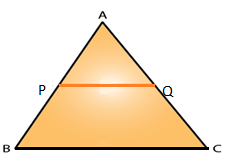It’s given that,

Ar(Δ APQ) = ½ Ar(Δ ABC)

Ar(Δ APQ)/ Ar(Δ ABC) = ½

AP2/ AB2 = ½

AP/ AB = 1/√2

(AB – BP)/ AB = 1/√2

1 – (BP/AB) = 1/√2

BP/AB = 1 – 1/√2

Thus,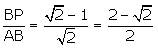[Multiplying by √2 in both numerator & denominator]

6. In the given triangle PQR, LM is parallel to QR and PM: MR = 3: 4.

Calculate the value of ratio: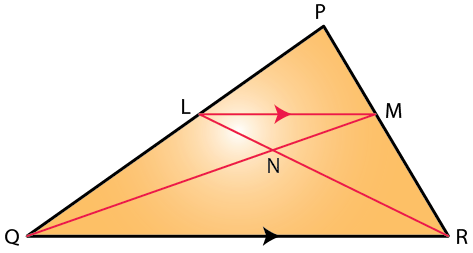(i) PL/PQ and then LM/QR

(ii) Area of Δ LMN/ Area of Δ MNR

(iii) Area of Δ LQM/ Area of Δ LQN

Solution:

(i) In Δ PLM and Δ PQR,

As LM || QR, corresponding angles are equal.

∠PLM = ∠PQR

∠PML = ∠PRQ

Hence, ∆PLM ~ ∆PQR by AA criterion for similarity.

So, we have

PM/ PR = LM/ QR

3/7 = LM/QR [Since, PM/MR = ¾ ⇒ PM/PR = 3/7]

And, by BPT we have

PL/LQ = PM/MR = ¾

LQ/PL = 4/3

1 + (LQ/PL) = 1 + 4/3

(PL + LQ)/ PL = (3 + 4)/3

PQ/PL = 7/3

Hence, PL/PQ = 3/7

(ii) As Δ LMN and Δ MNR have common vertex at M and their bases LN and NR are along the same straight line

Hence, Ar (Δ LMN)/ Ar (Δ RNQ) = LN/NR

Now, in Δ LMN and Δ RNQ we have,

∠NLM = ∠NRQ [Alternate angles]

∠LMN = ∠NQR [Alternate angles]

Thus, ∆LNM ~ ∆RNQ by AA criterion for similarity.

So,

MN/QN = LN/NR = LM/QR = 3/7

Therefore,

Ar (Δ LMN)/ Ar (Δ MNR) = LN/NR = 3/7

(iii) As Δ LQM and Δ LQN have common vertex at L and their bases QM and QN are along the same straight line.

Area of Δ LQM/ Area of Δ LQN = QM/QN = 10/7

[Since, MN/QN = 3/7 ⇒ QM/QN = 10/7]

### Exercise 15(D) Page No: 229

1. A triangle ABC has been enlarged by scale factor m = 2.5 to the triangle A’ B’ C’ Calculate:

(i) the length of AB, if A’ B’ = 6 cm.

(ii) the length of C’ A’ if CA = 4 cm.

Solution:

Given that, Δ ABC has been enlarged by scale factor m of 2.5 to Δ A’B’C’.

(i) A’B’ = 6 cm

So,

AB(2.5) = A’B’ = 6 cm

AB = 2.4 cm

(ii) CA = 4 cm

We know that,

CA(2.5) = C’A’

C’A’ = 4 x 2.5 = 10 cm

2. A triangle LMN has been reduced by scale factor 0.8 to the triangle L’ M’ N’. Calculate:

(i) the length of M’ N’, if MN = 8 cm.

(ii) the length of LM, if L’ M’ = 5.4 cm.

Solution:

Given, Δ LMN has been reduced by a scale factor m = 0.8 to Δ L’M’N’.

(i) MN = 8 cm

So, MN (0.8) = M’N’

(8)(0.8) = M’N’

M’N’ = 6.4 cm

(ii) L’M’ = 5.4 cm

So, LM (0.8) = L’M’

LM (0.8) = 5.4

LM = 6.75 cm

3. A triangle ABC is enlarged, about the point 0 as centre of enlargement, and the scale factor is 3. Find:

(i) A’B’, if AB = 4 cm.

(ii) BC, if B’C’ = 15 cm.

(iii) OA, if OA’ = 6 cm

(iv) OC’, if OC = 21 cm

Also, state the value of:

(a) OB’/OB (b) C’A’/CA

Solution:

Given that, Δ ABC is enlarged and the scale factor m = 3 to the Δ A’B’C’.

(i) AB = 4 cm

So, AB(3) = A’B’

(4)(3) = A’B’

A’B’ = 12 cm

(ii) B’C’ = 15 cm

So, BC(3) = B’C’

BC(3) = 15

BC = 5 cm

(iii) OA’ = 6 cm

So, OA (3) = OA’

OA (3) = 6

OA = 2 cm

(iv) OC = 21 cm

So, OC(3) = OC’

21 x 3 = OC’

OC’ = 63 cm

The ratio of the lengths of the two corresponding sides of two triangles.

Δ ABC is enlarged and the scale factor m = 3 to the Δ A’B’C’

Hence,

(a) OB’/OB = 3

(b) C’A’/CA = 3

### Exercise 15(E) Page No: 229

1. In the following figure, XY is parallel to BC, AX = 9 cm, XB = 4.5 cm and BC = 18 cm.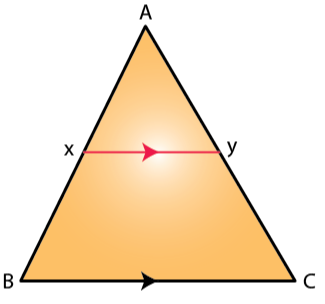Find:

(i) AY/YC (ii) YC/AC (iii) XY

Solution:

Given, XY || BC.

So, In Δ AXY and Δ ABC

∠AXY = ∠ABC [Corresponding angles]

∠AYX = ∠ACB [Corresponding angles]

Hence, ∆AXY ~ ∆ABC by AA criterion for similarity.

As corresponding sides of similar triangles are proportional, we have

(i) AX/AB = AY/AC

9/13.5 = AY/AC

AY/YC = 9 / 4.5

AY/YC = 2

AY/YC = 2/1

(ii) We have,

AX/AB = AY/AC

9/13.5 = AY/ AC

YC/AC = 4.5/13.5 = 1/3

(iii) As, ∆AXY ~ ∆ABC

AX/AB = XY/BC

9/13.5 = XY/18

XY = (9 x 18)/ 13.5 = 12 cm

2. In the following figure, ABCD to a trapezium with AB ‖ DC. If AB = 9 cm, DC = 18 cm, CF= 13.5 cm, AP = 6 cm and BE = 15 cm,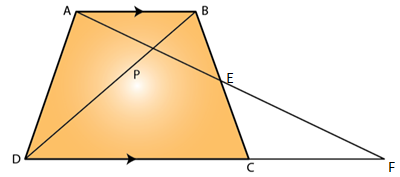Calculate:

(i) EC (ii) AF (iii) PE

Solution:

(i) In Δ AEB and Δ FEC,

∠AEB = ∠FEC [Vertically opposite angles]

∠BAE = ∠CFE [Since, AB||DC]

Hence, ∆AEB ~ ∆FEC by AA criterion for similarity.

So, we have

AE/FE = BE/EC = AB/FC

15/EC = 9/13.5

EC = 22.5 cm

(ii) In Δ APB and Δ FPD,

∠APB = ∠FPD [Vertically opposite angles]

∠BAP = ∠DFP [Since, AB||DF]

Hence, ∆APB ~ ∆FPD by AA criterion for similarity.

So, we have

AP/FP = AB/FD

6/FP = 9/31.5

FP = 21 cm

So, AF = AP + PF = 6 + 21 = 27 cm

(iii) We already have, ∆AEB ~ ∆FEC

So,

AE/FE = BE/CE = AB/FC

AE/FE = 9/13.5

(AF – EF)/ FE = 9/13.5

AF/EF – 1 = 9/13.5

27/EF = 9/13.5 + 1 = 22.5/ 13.5

EF = (27 x 13.5)/22.5 = 16.2 cm

Now, PE = PF – EF = 21 – 16.2 = 4.8 cm

3. In the following figure, AB, CD and EF are perpendicular to the straight line BDF.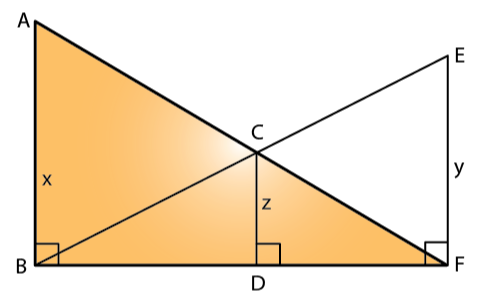If AB = x and; CD = z unit and EF = y unit, prove that:

1/x + 1/y = 1/z

Solution:

In Δ FDC and Δ FBA,

∠FDC = ∠FBA [As DC || AB]

∠DFC = ∠BFA [common angle]

Hence, ∆FDC ~ ∆FBA by AA criterion for similarity.

So, we have

DC/AB = DF/BF

z/x = DF/BF …. (1)

In Δ BDC and Δ BFE,

∠BDC = ∠BFE [As DC || FE]

∠DBC = ∠FBE [Common angle]

Hence, ∆BDC ~ ∆BFE by AA criterion for similarity.

So, we have

BD/BF = z/y ….. (2)

Now, adding (1) and (2), we get

BD/BF + DF/BF = z/y + z/x

1 = z/y + z/x

Thus,

1/z = 1/x + 1/y

– Hence Proved

4. Triangle ABC is similar to triangle PQR. If AD and PM are corresponding medians of the two triangles, prove that: AB/PQ = AD/PM.

Solution: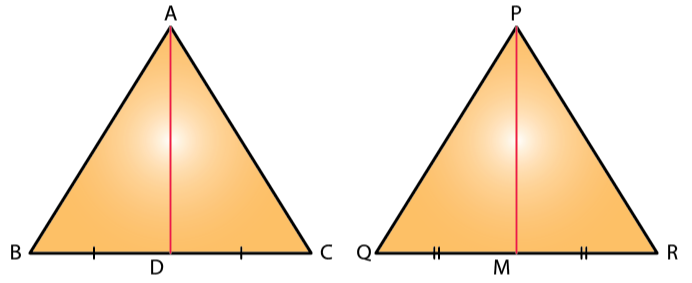Given, ∆ABC ~ ∆PQR

AD and PM are the medians, so BD = DC and QM = MR

So, we have

AB/PQ = BC/QR [Corresponding sides of similar triangles are proportional.]

Then,

AB/PQ = (BC/2)/ (QR/2) = BD/QM

And, ∠ABC = ∠PQR i.e. ∠ABD = ∠PQM

Hence, ∆ABD ~ ∆PQM by SAS criterion for similarity.

Thus,

5. Triangle ABC is similar to triangle PQR. If AD and PM are altitudes of the two triangles, prove that: AB/PQ = AD/PM.

Solution: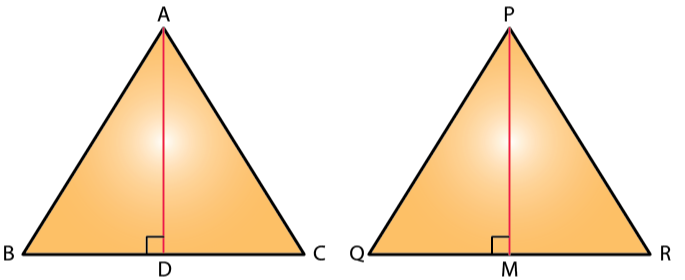Given, ∆ABC ~ ∆PQR

So,

∠ABC = ∠PQR i.e. ∠ABD = ∠PQM

Also, ∠ADB = ∠PMQ [Both are right angles]

Hence, ∆ABD ~ ∆PQM by AA criterion for similarity.

Thus,

6. Triangle ABC is similar to triangle PQR. If bisector of angle BAC meets BC at point D and bisector of angle QPR meets QR at point M, prove that: AB/PQ = AD/PM

Solution: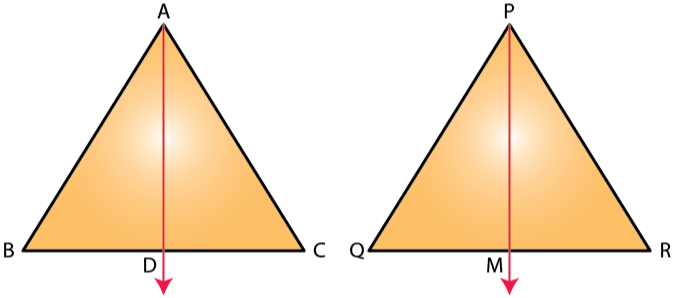Given, ∆ABC ~ ∆PQR

And, AD and PM are the angle bisectors.

So,

Also, ∠ABC = ∠PQR i.e. ∠ABD = ∠PQM

Hence, ∆ABD ~ ∆PQM by AA criterion for similarity.

Thus,

7. In the following figure, ∠AXY = ∠AYX. If BX/AX = CY/AY, show that triangle ABC is isosceles.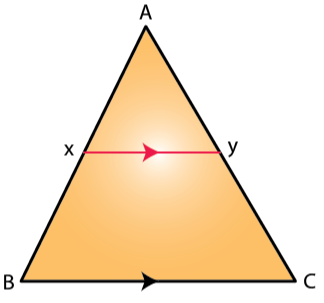Solution:

Given,

∠AXY = ∠AYX

So, AX = AY [Sides opposite to equal angles are equal.]

Also, from BPT we have

BX/AX = CY/AY

Thus,

AX + BX = AY + CY

So, AB = AC

Therefore, ∆ABC is an isosceles triangle.

8. In the following diagram, lines l, m and n are parallel to each other. Two transversals p and q intersect the parallel lines at points A, B, C and P, Q, R as shown.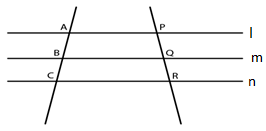Prove that: AB/BC = PQ/QR

Solution: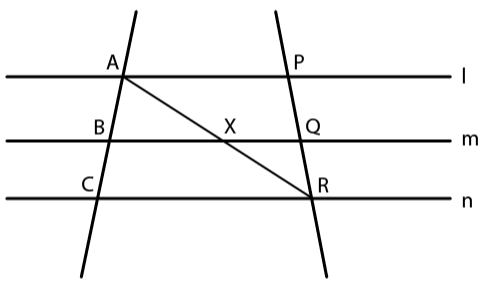Let join AR such that it intersects BQ at point X.

In ∆ACR, BX || CR. By BPT, we have

AB/BC = AX/XR … (1)

In ∆APR, XQ || AP. By BPT, we have

PQ/QR = AX/XR … (2)

From (1) and (2),

AB/BC = PQ/QR

– Hence Proved

9. In the following figure, DE || AC and DC || AP. Prove that: BE/EC = BC/CP.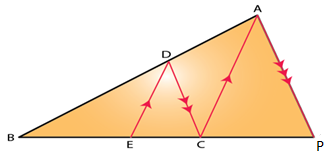Solution:

Given, DE || AC

So,

BE/EC = BD/DA [By BPT]

And, DC || AP

So,

BC/CP = BD/DA [By BPT]

Therefore,

BE/EC = BC/CP

10. In the figure given below, AB ‖ EF ‖ CD. If AB = 22.5 cm, EP = 7.5 cm, PC = 15 cm and DC = 27 cm.

Calculate: (i) EF (ii) AC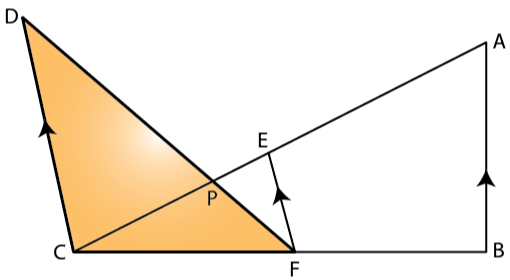Solution:

(i) In ∆PCD and ∆PEF,

∠CPD = ∠EPF [Vertically opposite angles]

∠DCE = ∠FEP [As DC || EF, alternate angles.]

Hence, ∆PCD ~ ∆PEF by AA criterion for similarity.

So, we have

27/EF = 15/7.5

Thus,

EF = 13.5

(ii) And, as EF || AB

∆CEF ~ ∆CAB by AA criterion for similarity.

EC/AC = EF/AB

22.5/AC = 13.5/22.5

Thus, AC = 37.5 cm

11. In ΔABC, ∠ABC = ∠DAC, AB = 8 cm, AC = 4 cm and AD = 5 cm.

(i) Prove that ΔACD is similar to ΔBCA.

(ii) Find BC and CD

(iii) Find the area of ΔACD: area of ΔABC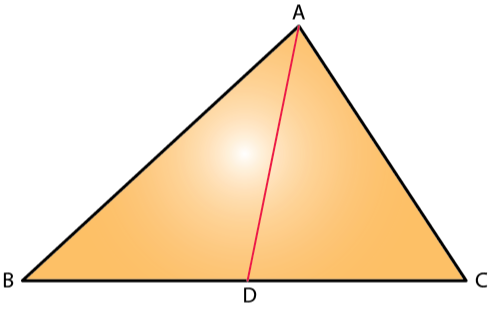Solution:

(i) In ∆ACD and ∆BCA,

∠DAC = ∠ABC [Given]

∠ACD = ∠BCA [Common angles]

Hence, ∆ACD ~ ∆BCA by AA criterion for similarity.

(ii) Since, ∆ACD ~ ∆BCA

We have,

4/BC = CD/4 = 5/8

4/BC = 5/8

So, BC = 32/5 = 6.4 cm

And,

CD/4 = 5/8

Thus, CD = 20/8 = 2.5 cm

(iii) As, ∆ACD ~ ∆BCA

We have,

Ar(∆ACD)/ Ar(∆BCA) = AD2/ AB2 = 52/82

Ar(∆ACD)/ Ar(∆BCA) = 25/64

12. In the given triangle P, Q and R are mid-points of sides AB, BC and AC respectively. Prove that triangle QRP is similar to triangle ABC.

Solution: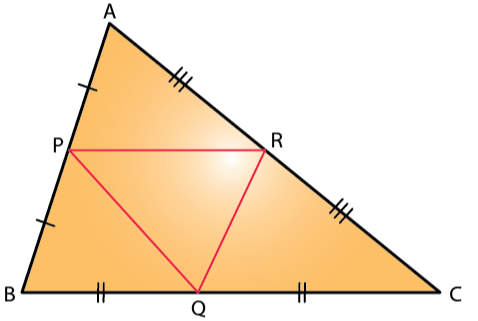In ∆ABC, as PR || BC by BPT we have

AP/PB = AR/RC

And, in ∆PAR and ∆BAC,

∠PAR = ∠BAC [Common]

∠APR = ∠ABC [Corresponding angles]

Hence, ∆PAR ~ ∆BAC by AA criterion for similarity

So, we have

PR/BC = AP/AB

PR/BC = ½ [Since, P is the mid-point of AB]

PR = ½ BC

Similarly,

PQ = ½ AC

RQ = ½ AB

So,

PR/BC = PQ/AC = RQ/AB

Therefore,

∆QRP ~ ∆ABC by SSS similarity.

13. In the following figure, AD and CE are medians of ∆ABC. DF is drawn parallel to CE. Prove that: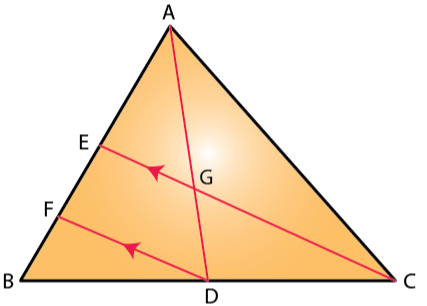(i) EF = FB,

(ii) AG: GD = 2: 1

Solution:

(i) In ∆BFD and ∆BEC,

∠BFD = ∠BEC [Corresponding angles]

∠FBD = ∠EBC [Common]

Hence, ∆BFD ~ ∆BEC by AA criterion for similarity.

So,

BF/BE = BD/BC

BF/BE = ½ [Since, D is the mid-point of BC]

BE = 2BF

BF = FE = 2BF

Thus,

EF = FB

(ii) In ∆AFD, EG || FD and using BPT we have

AE/EF = AG/GD …. (1)

Now, AE = EB [Since, E is the mid-point of AB]

AE = 2EF [As, EF = FB, by (1)]

So, from (1) we have

AG/GD = 2/1

Therefore, AG: GD = 2: 1

14. Two similar triangles are equal in area. Prove that the triangles are congruent.

Solution:

Let’s consider two similar triangles as ∆ABC ~ ∆PQR

So,

Ar(∆ABC)/ Ar(∆PQR) = (AB/PQ)2 = (BC/QR)2 = (AC/PR)2

Since,

Area of ∆ABC = Area of ∆PQR [Given]

Hence,

AB = PQ

BC = QR

AC = PR

So, as the respective sides of two similar triangles are all of same length.

We can conclude that,

∆ABC ≅ ∆PQR [By SSS rule]

– Hence Proved

15. The ratio between the altitudes of two similar triangles is 3: 5; write the ratio between their:

(i) medians. (ii) perimeters. (iii) areas.

Solution:

The ratio between the altitudes of two similar triangles is same as the ratio between their sides.

So,

(i) The ratio between the medians of two similar triangles is same as the ratio between their sides.

Thus, the required ratio = 3: 5

(ii) The ratio between the perimeters of two similar triangles is same as the ratio between their sides.

Thus, the required ratio = 3: 5

(iii) The ratio between the areas of two similar triangles is same as the square of the ratio between their corresponding sides.

Thus, the required ratio = (3)2: (5)2 = 9: 25

### Exercises of Selina Solutions Concise Maths Class 10 Chapter 15 Similarity (With Applications to Maps and Models)

Exercise 15(A) Solutions

Exercise 15(B) Solutions

Exercise 15(C) Solutions

Exercise 15(D) Solutions

Exercise 15(E) Solutions